# ICSE Selina Solution for Class 9 Chemistry Chapter 4: Atomic Structure and Chemical Bonding

## ICSE Selina Solution for Class 9 Chemistry Chapter 4 – Free PDF Download

ICSE Selina Solution for Class 9 Chemistry Chapter 4 Atomic Structure and Chemical Bonding provided here guides students in their ICSE Class 9 examination. This study material assists students in understanding the concepts completely by providing an insightful answer to all the exercise questions provided in ICSE Class 9 Selina publications textbook.

Selina Solutions for class 9 Chemistry is an important resource material since it deals with the basic topics of atomic structure and chemical bonding. This chapter is very critical to understand the Physical Chemistry topics in your higher studies.

ICSE Concise solutions provided here sheds light on the structure of an atom, mass number, atomic number, Octet rule. This solution helps you to understand constituents of atoms, electron distribution in orbits, electrovalent and covalent bonding.

### Important topics covered in ICSE Selina Solution for Class 9 Chemistry Chapter 4

• Definition of an Atom
• Discovery of an atom
• Discovery of Protons
• Discovery of Nucleus
• Bohr’s Atomic Model
• Discovery of Neutrons
• Structure of Atom
• Atomic Number
• Distribution of Electrons in the Orbits- Bohr-Bury Scheme
• Valence Electrons
• Reason for the chemical activity of an Atom
• Isotopes
• Ionic Electrovalent) Bond
• Covalent (Molecular) Bond

### Download PDF of ICSE Selina Solution for Class 9 Chemistry Chapter 4 Atomic Structure and Chemical Bonding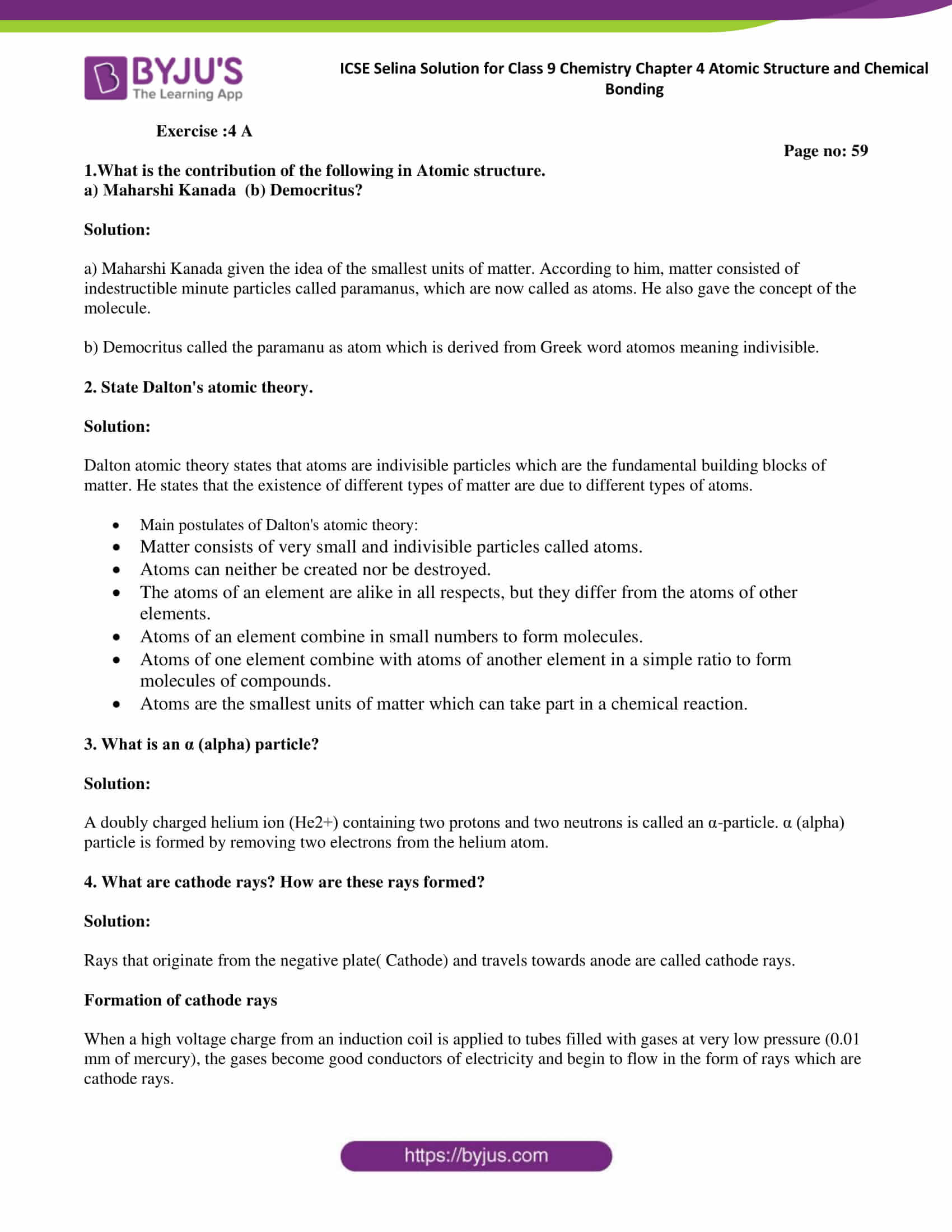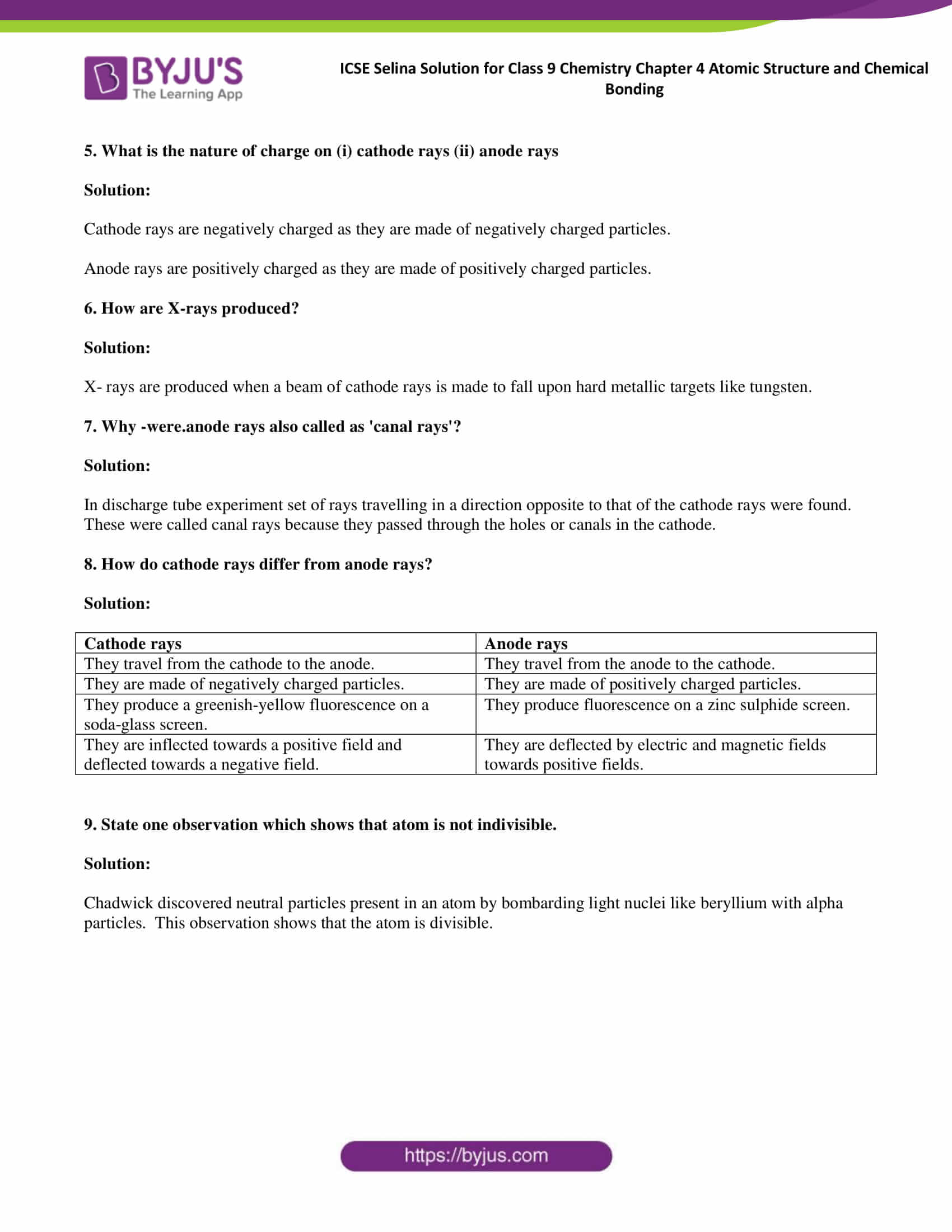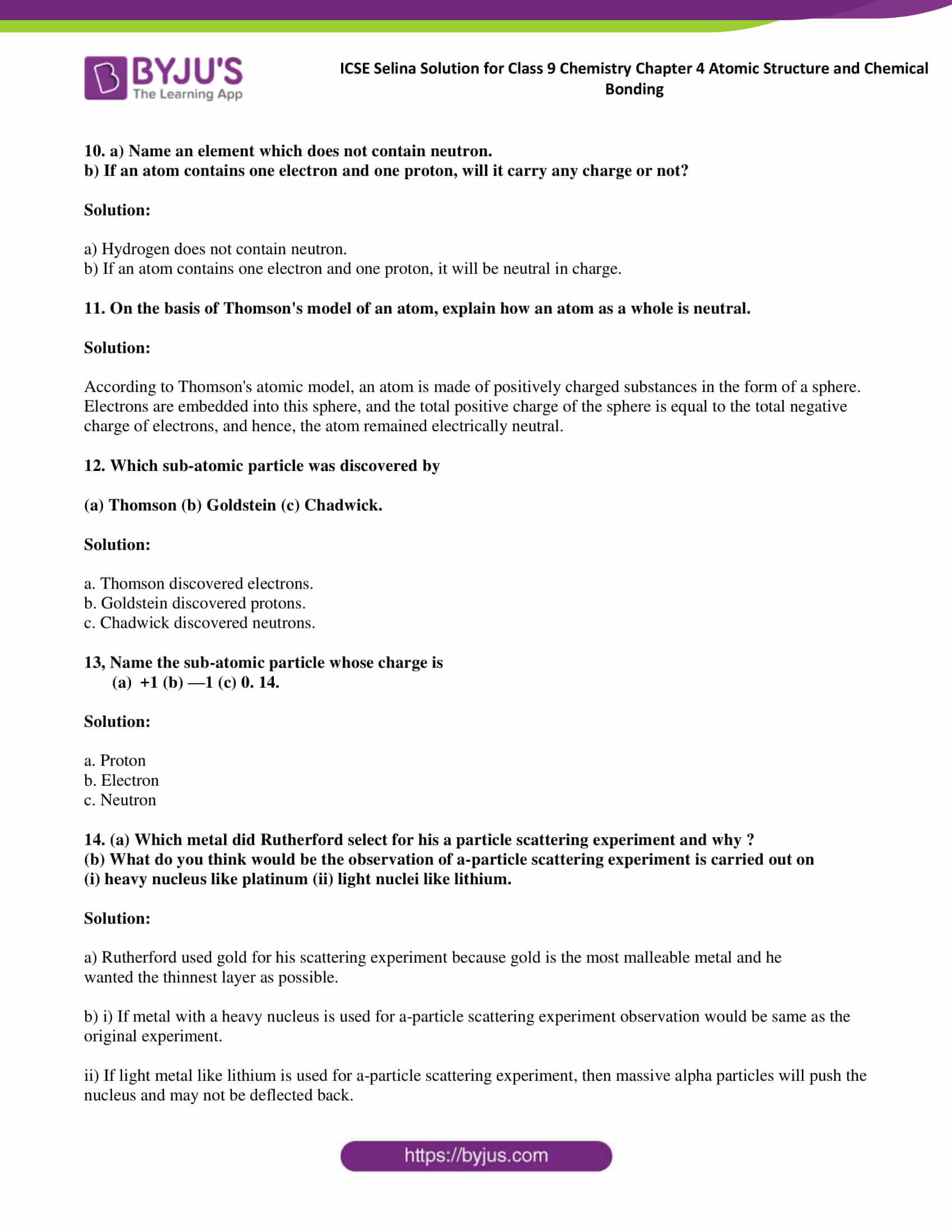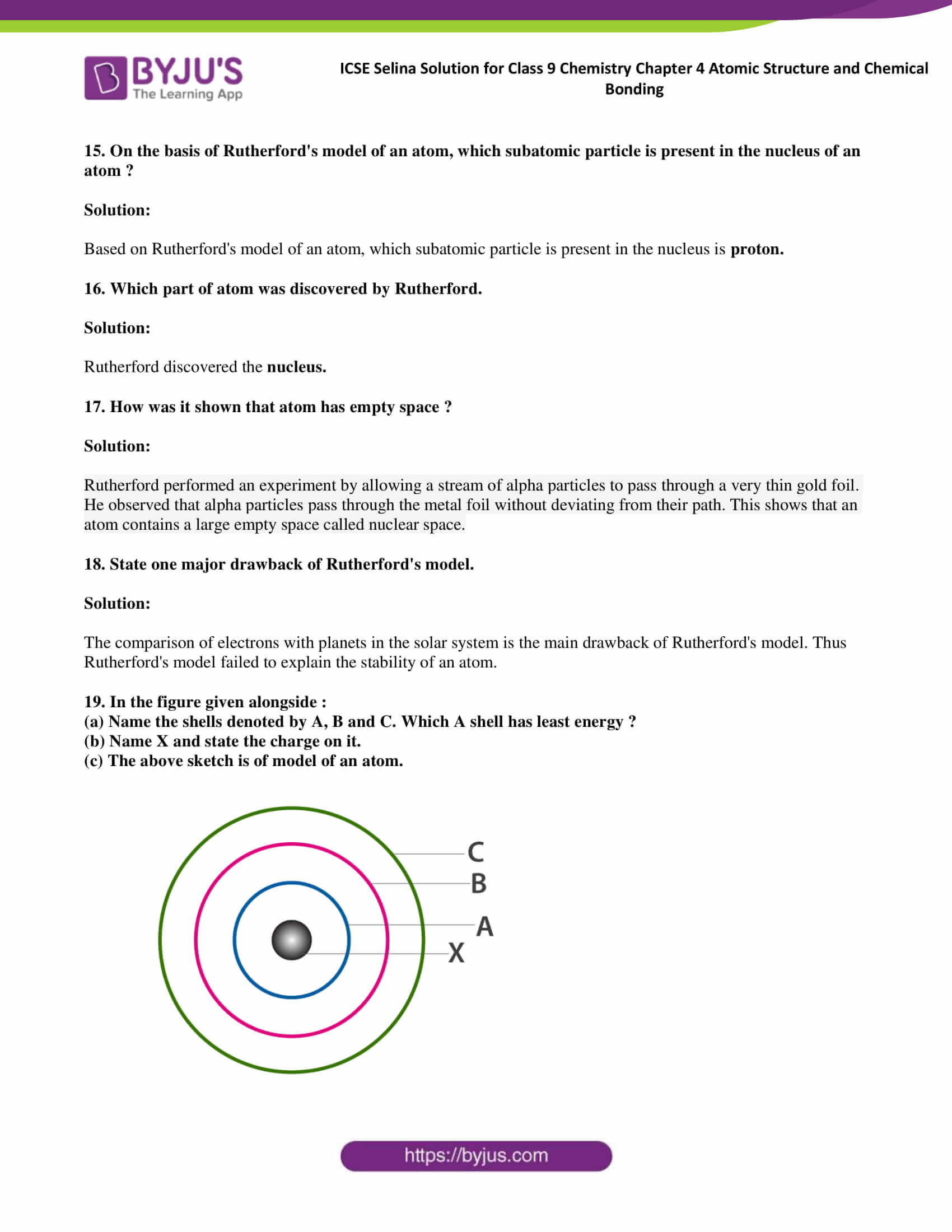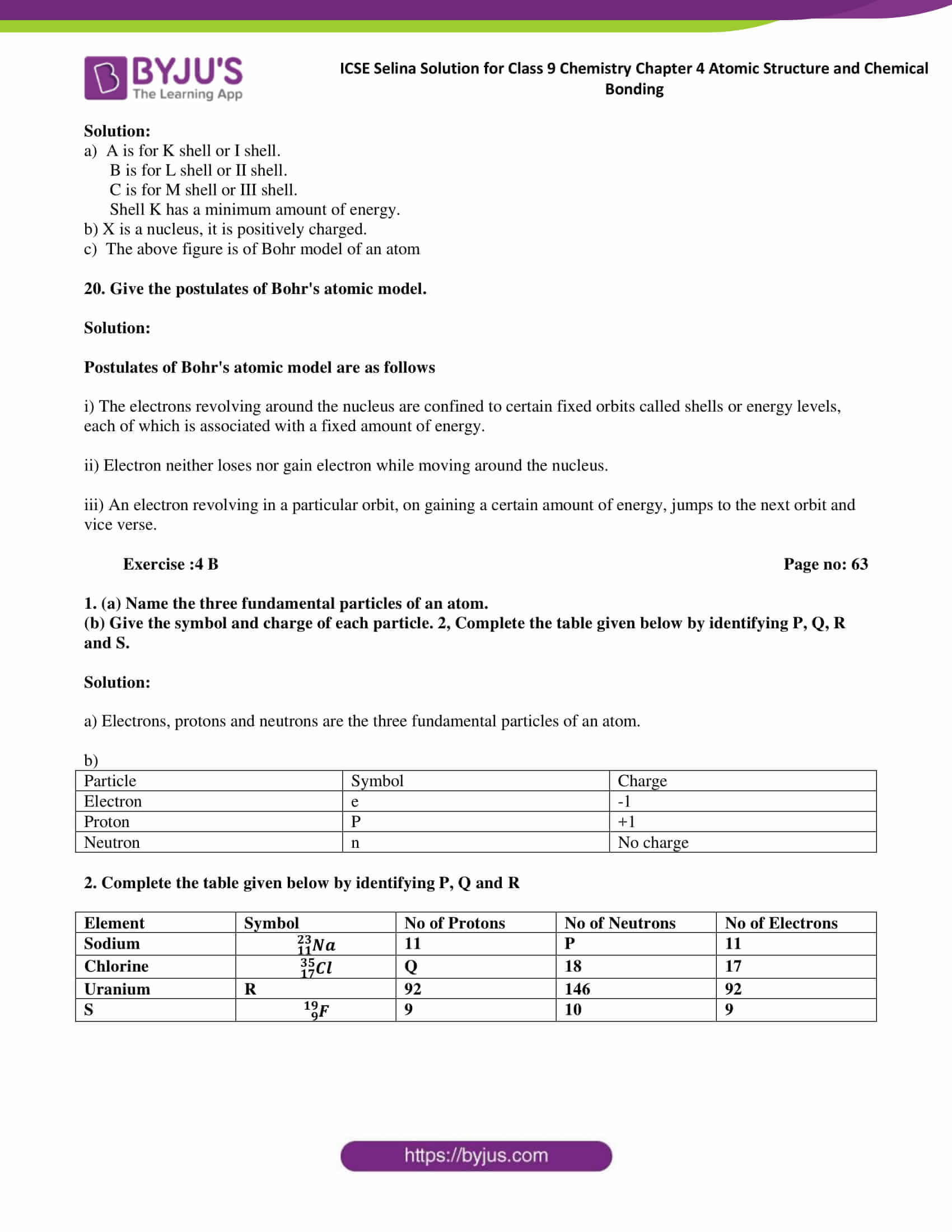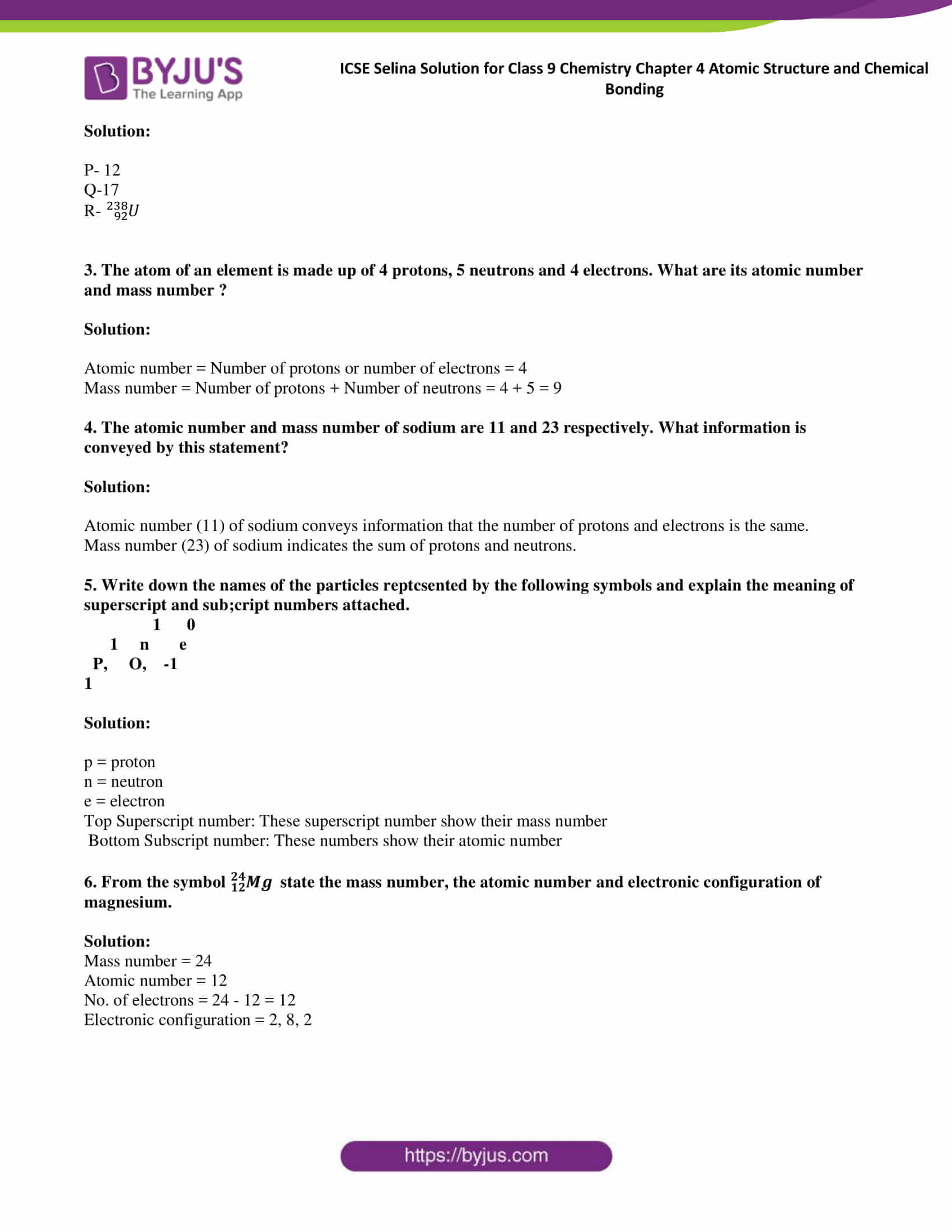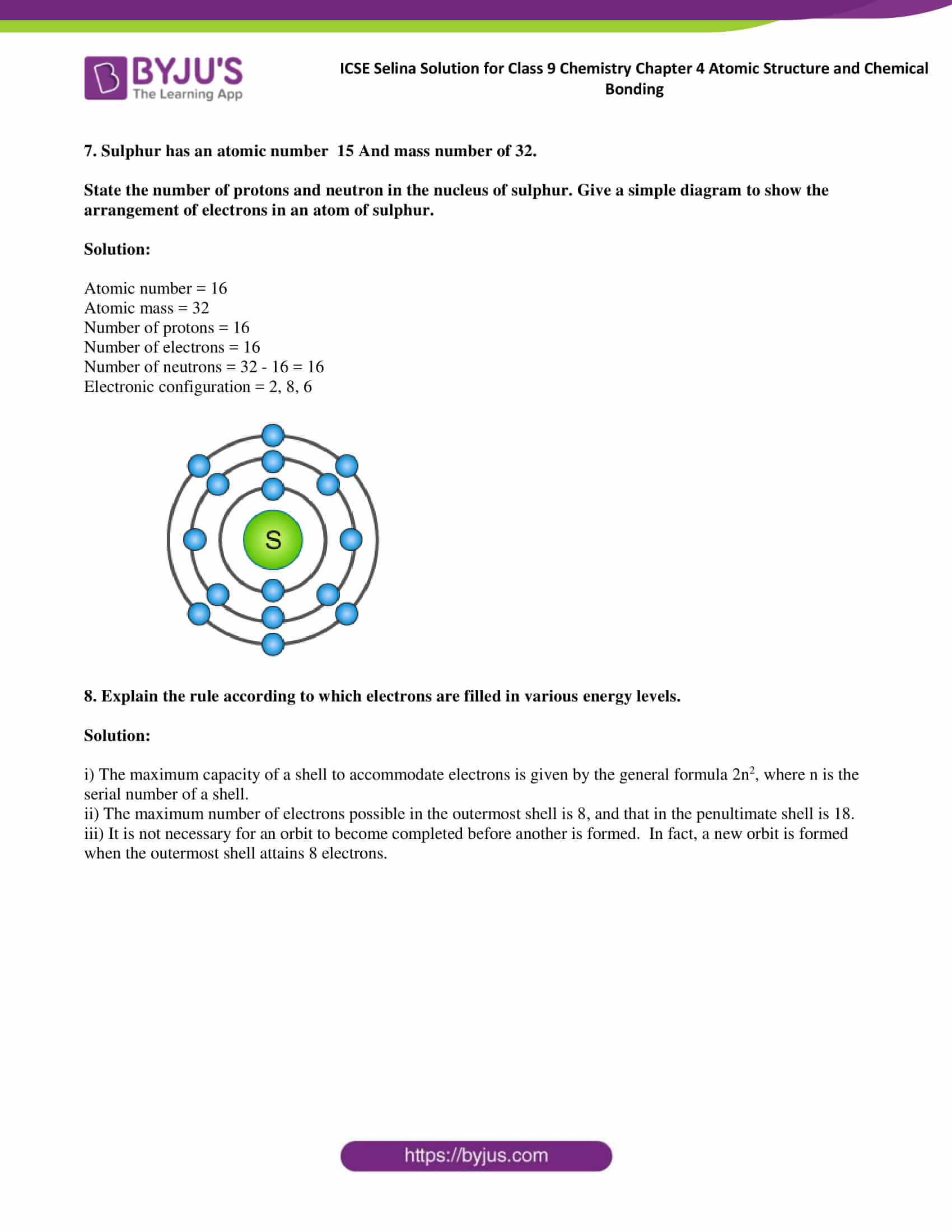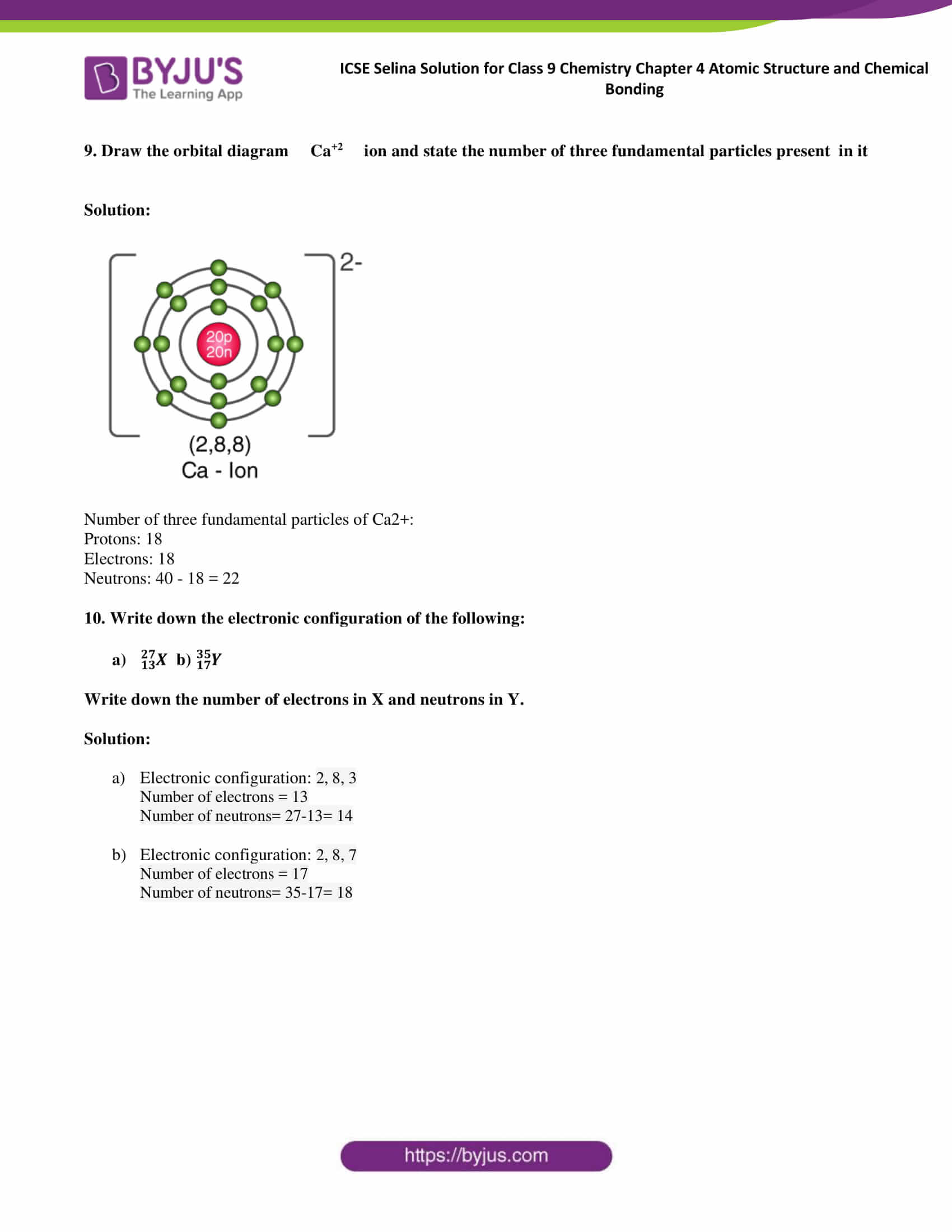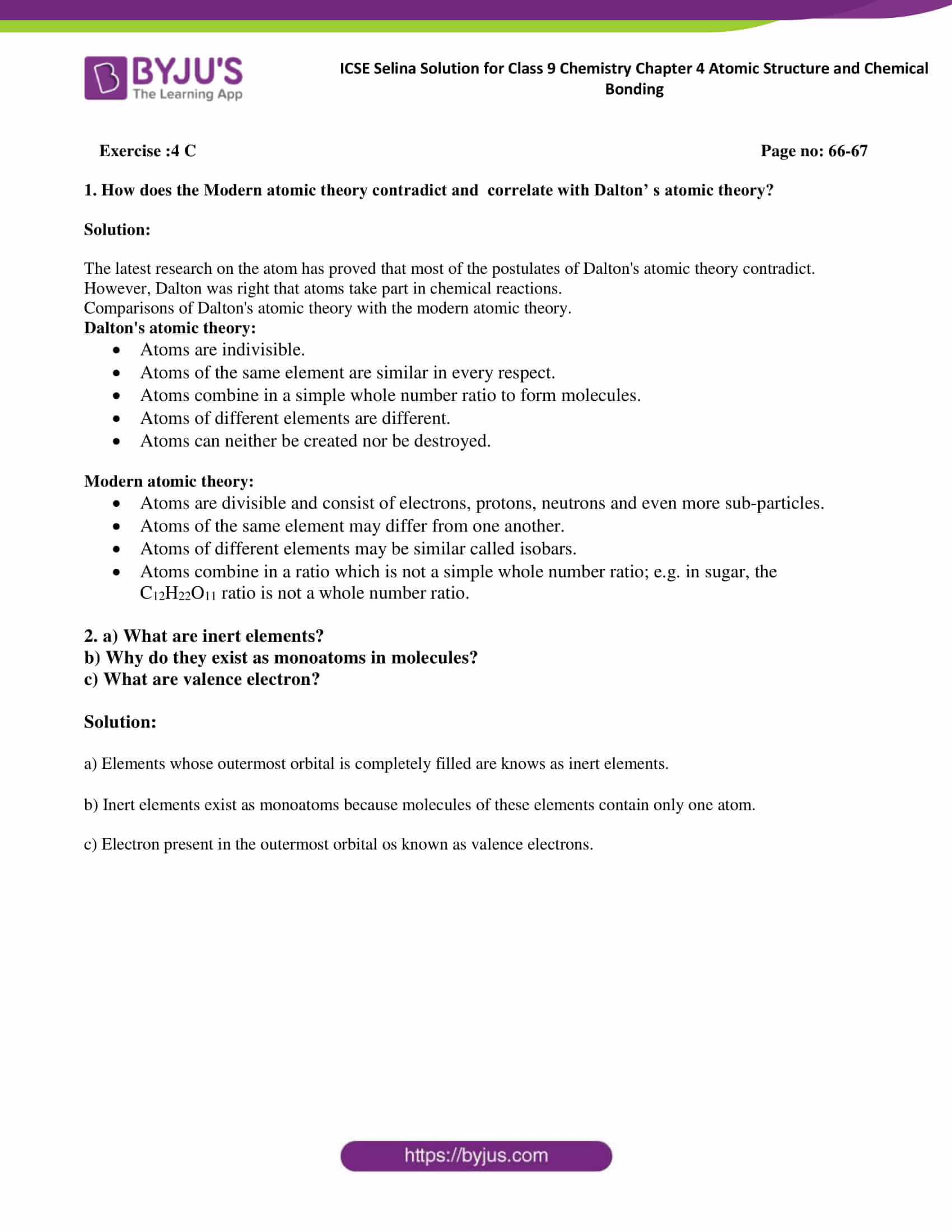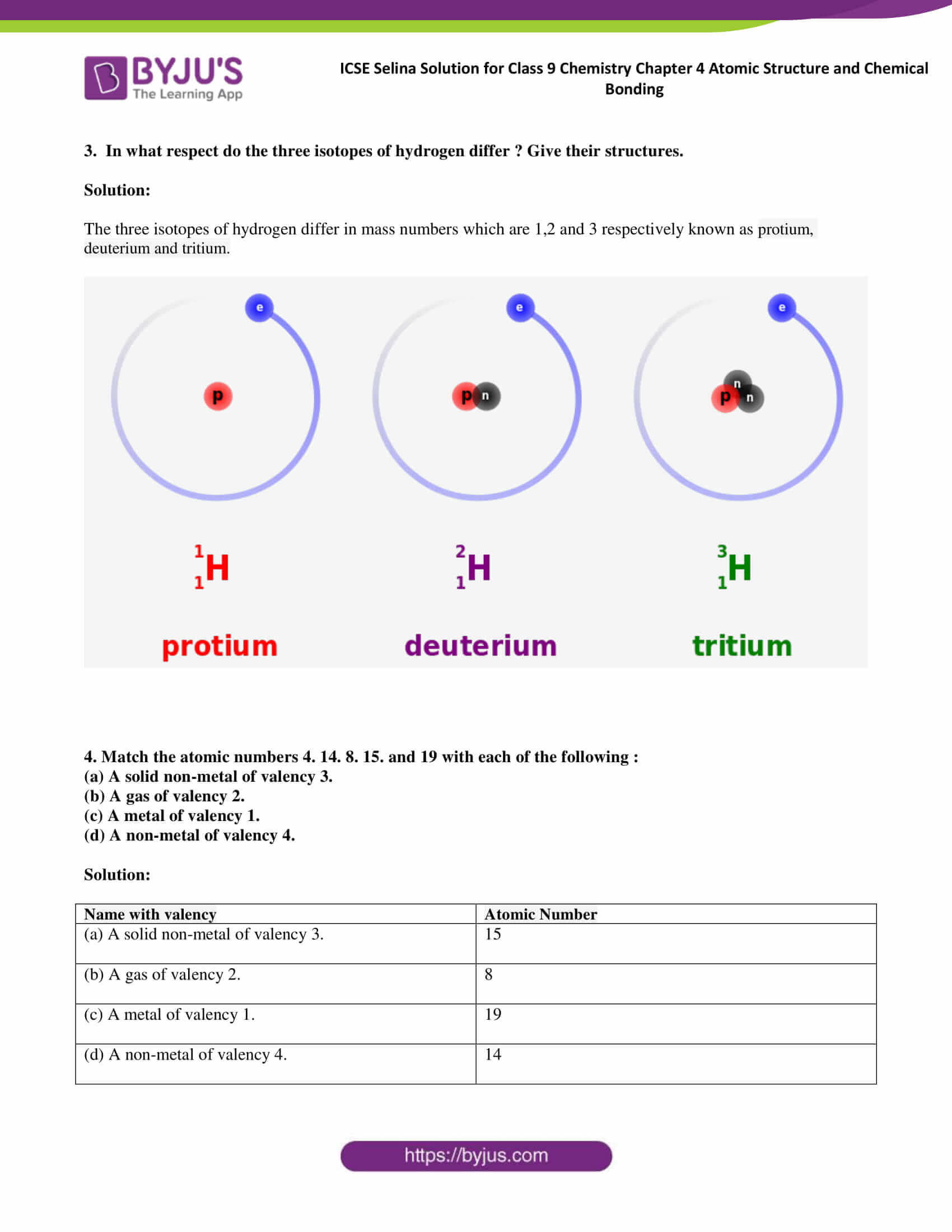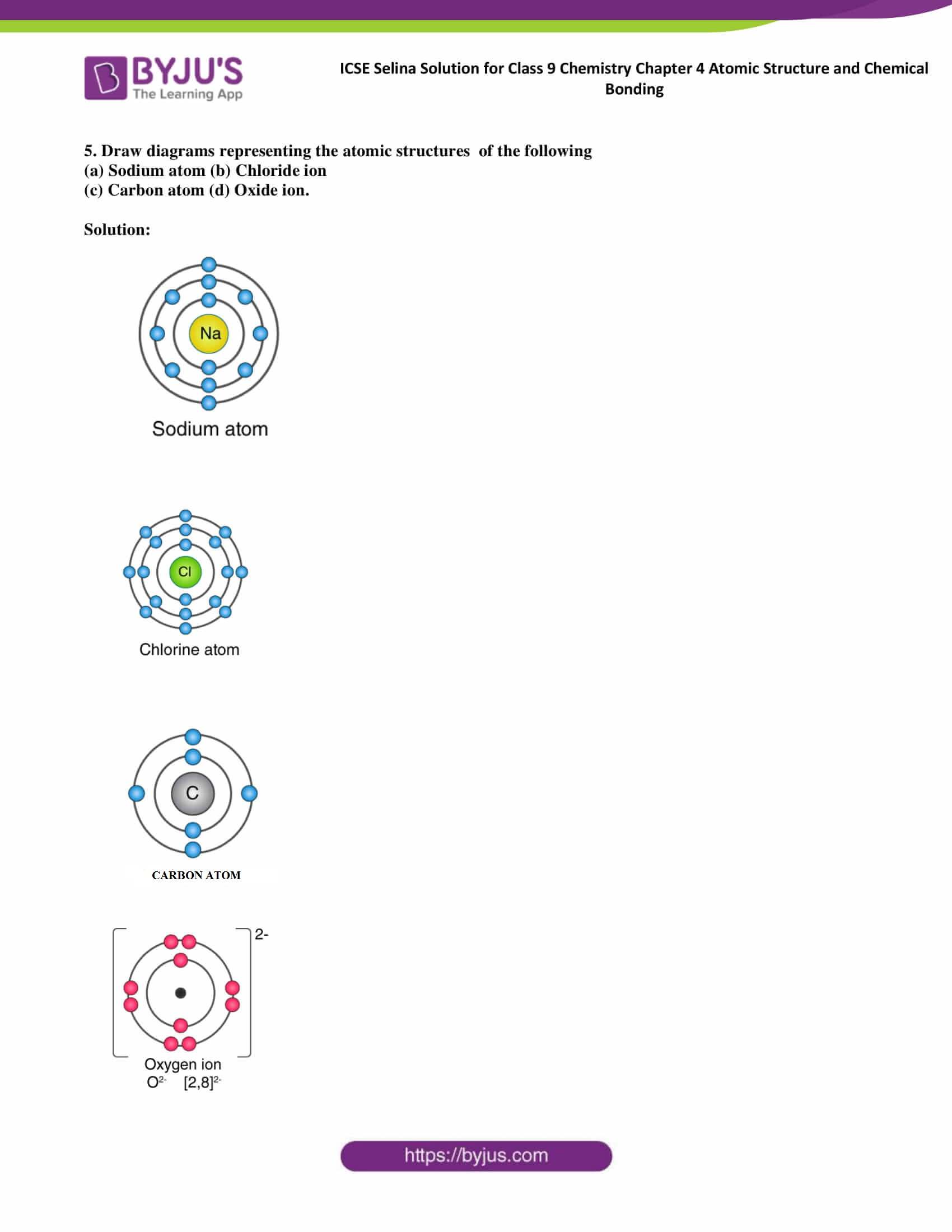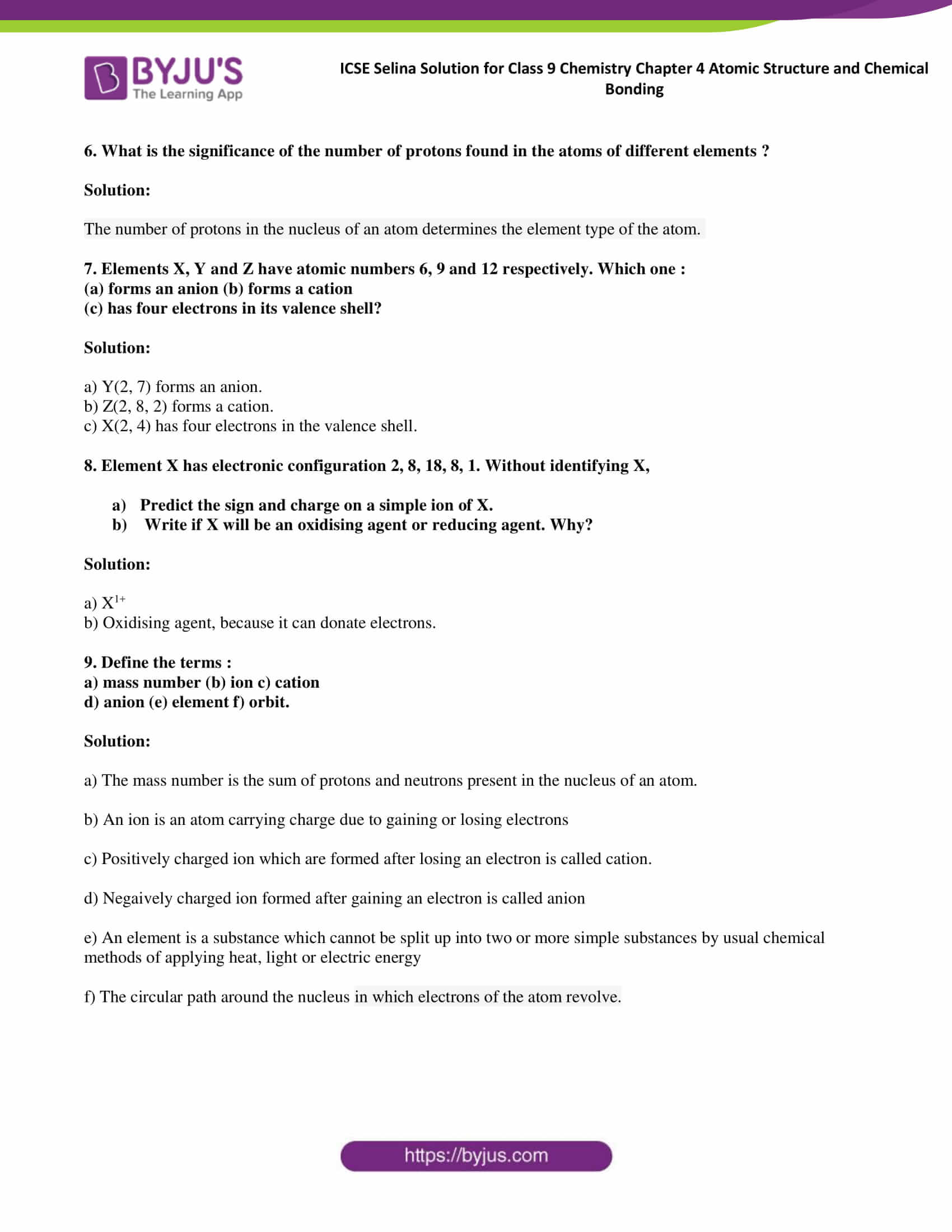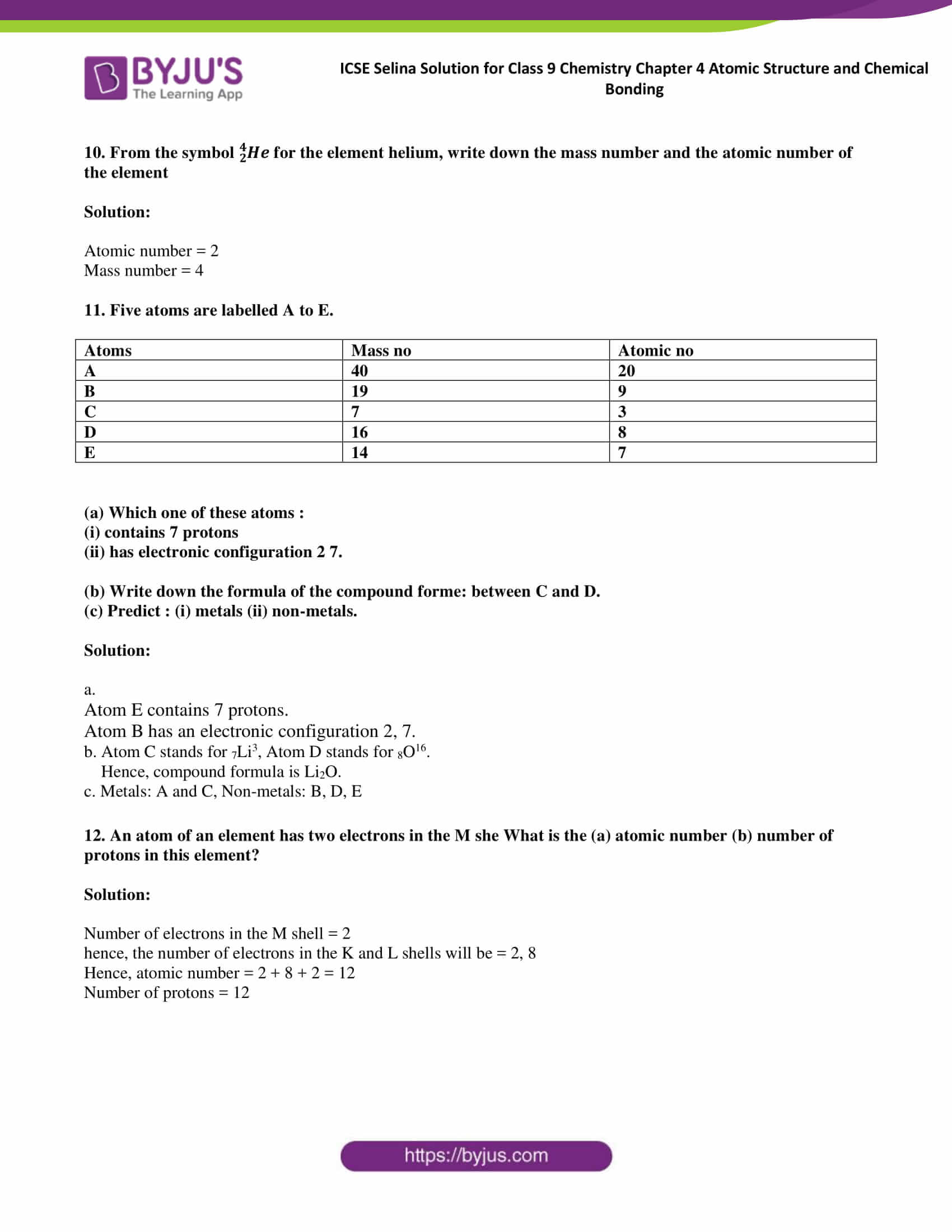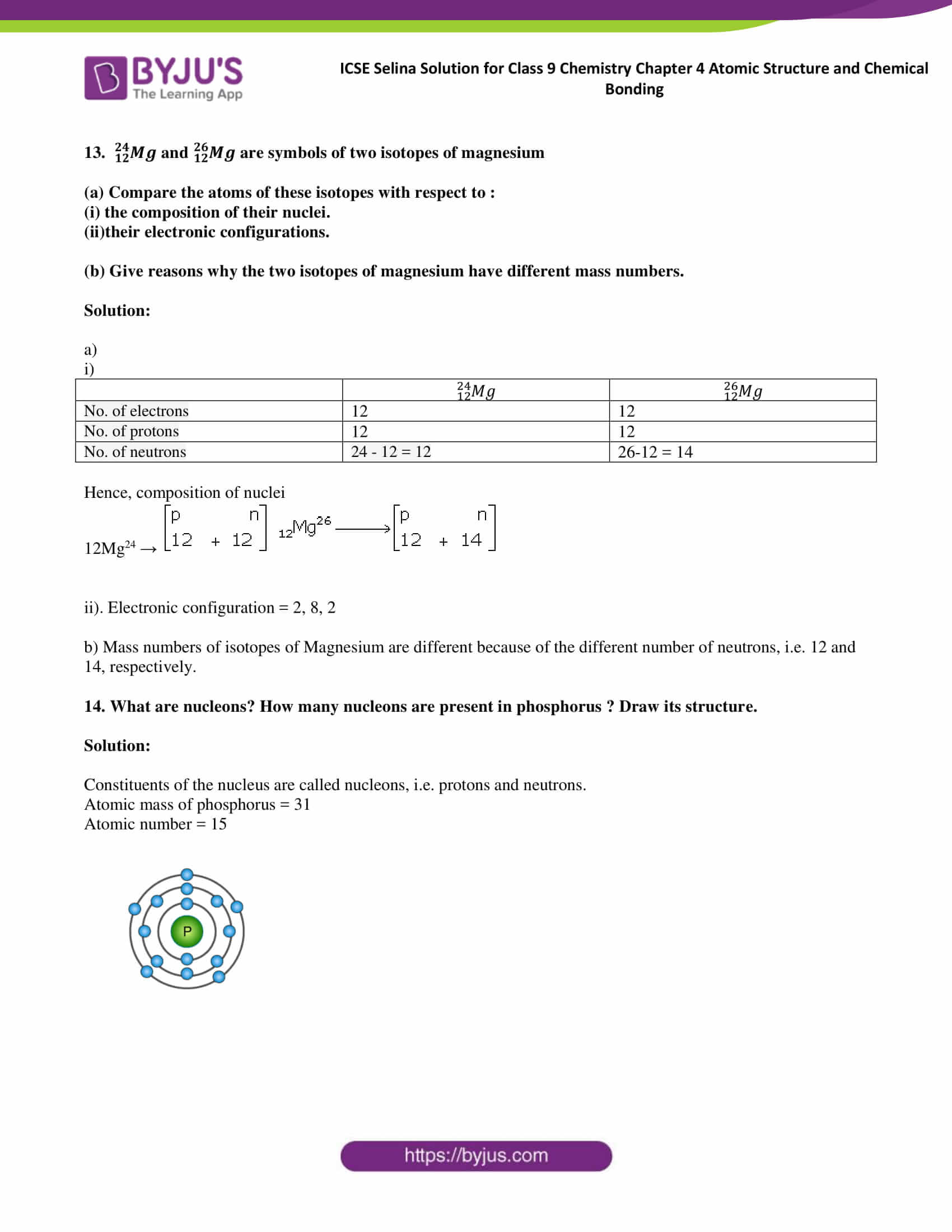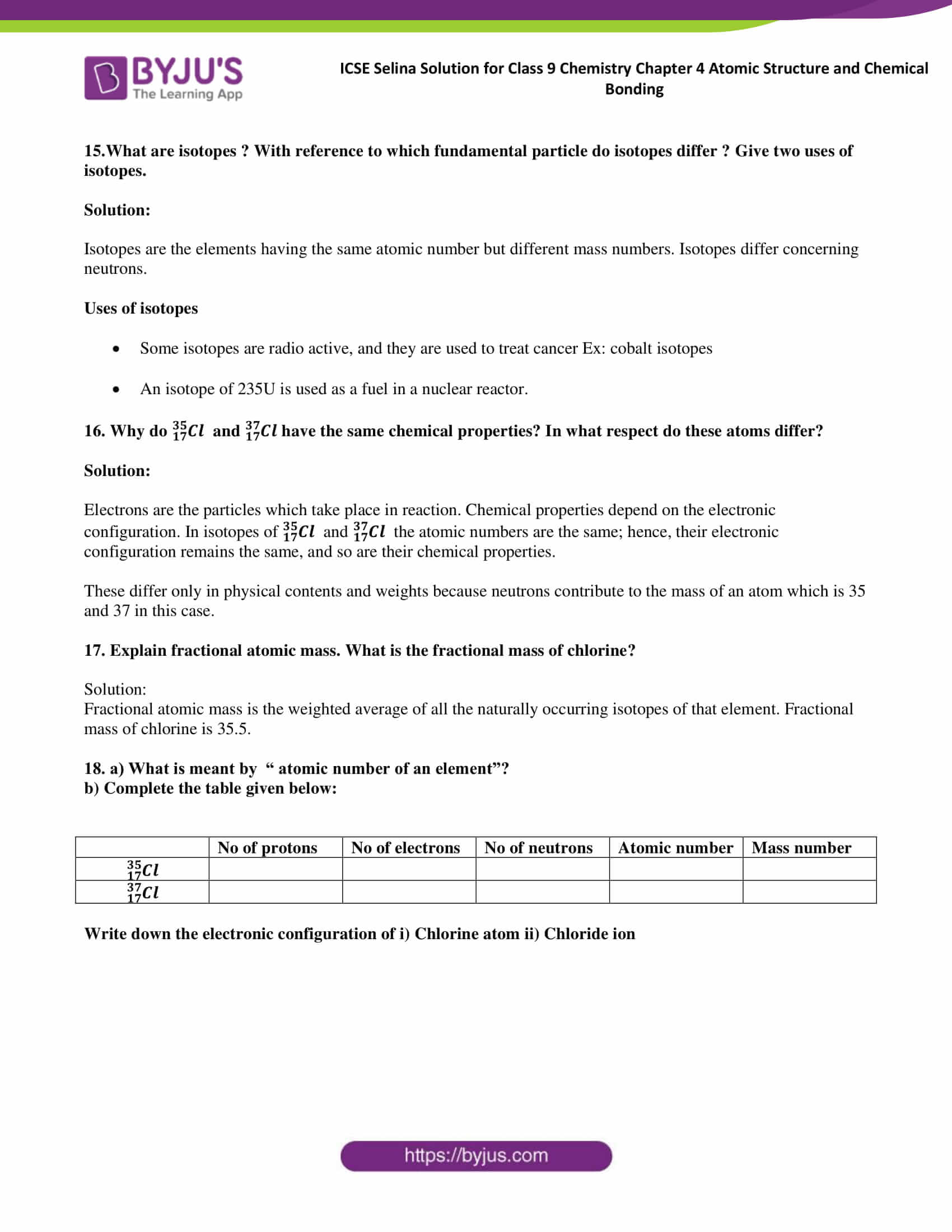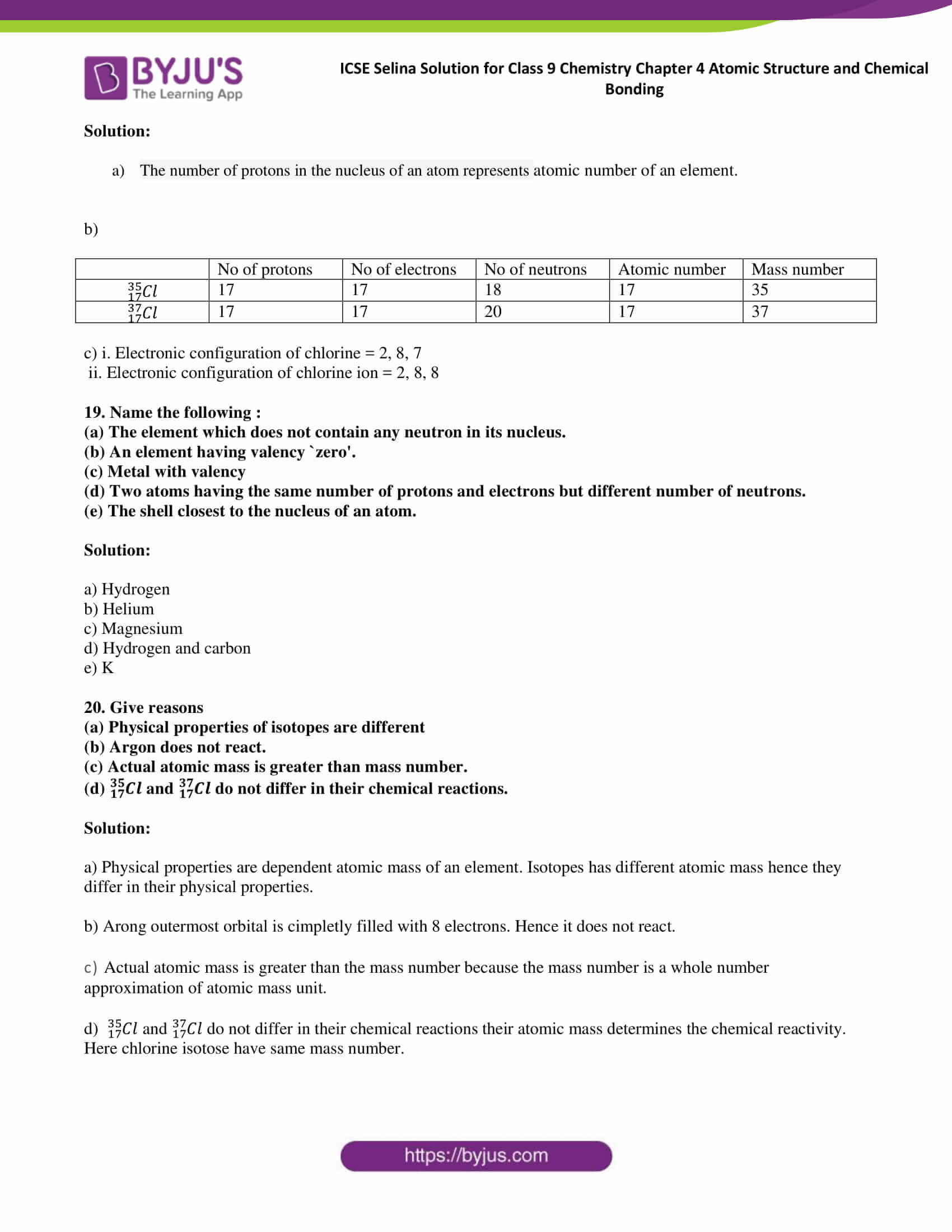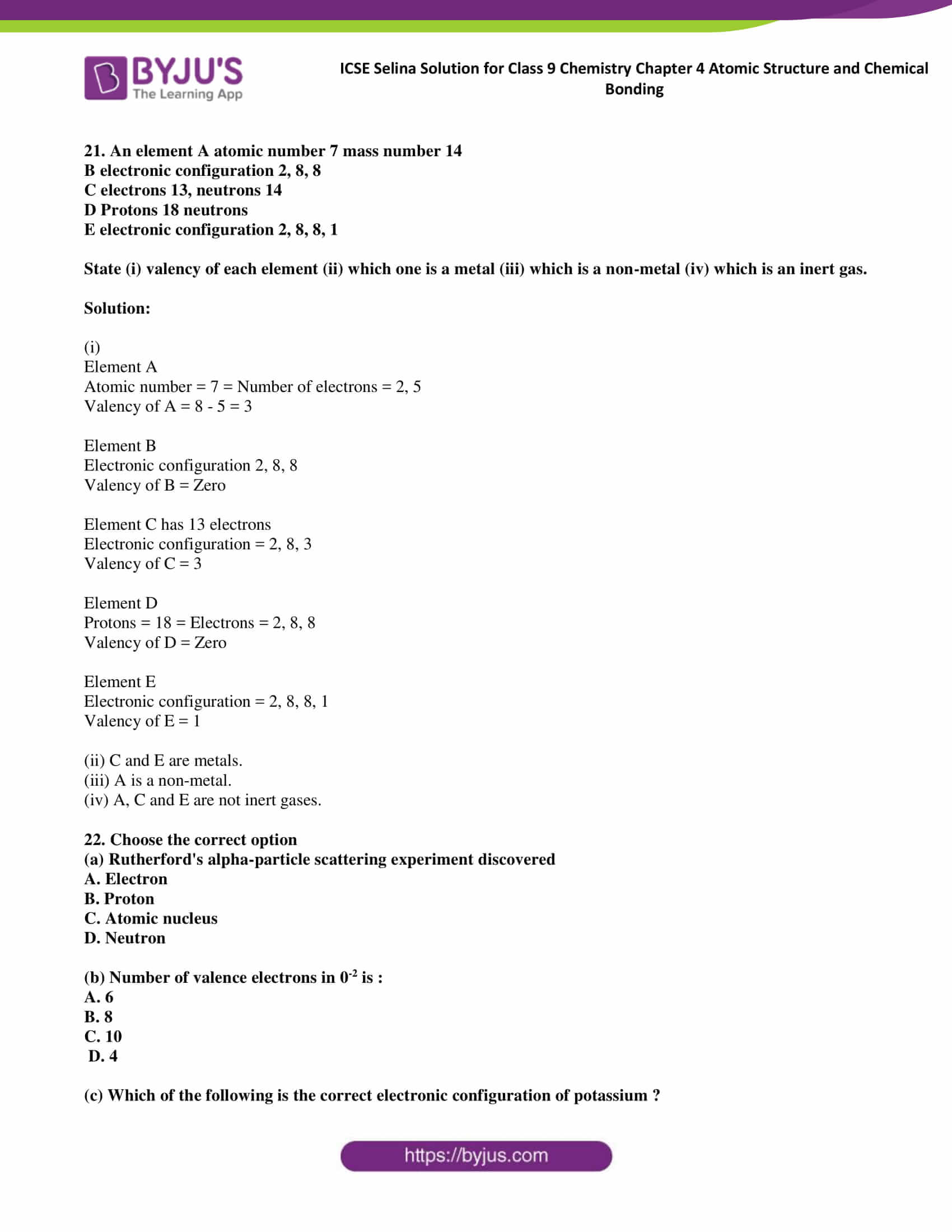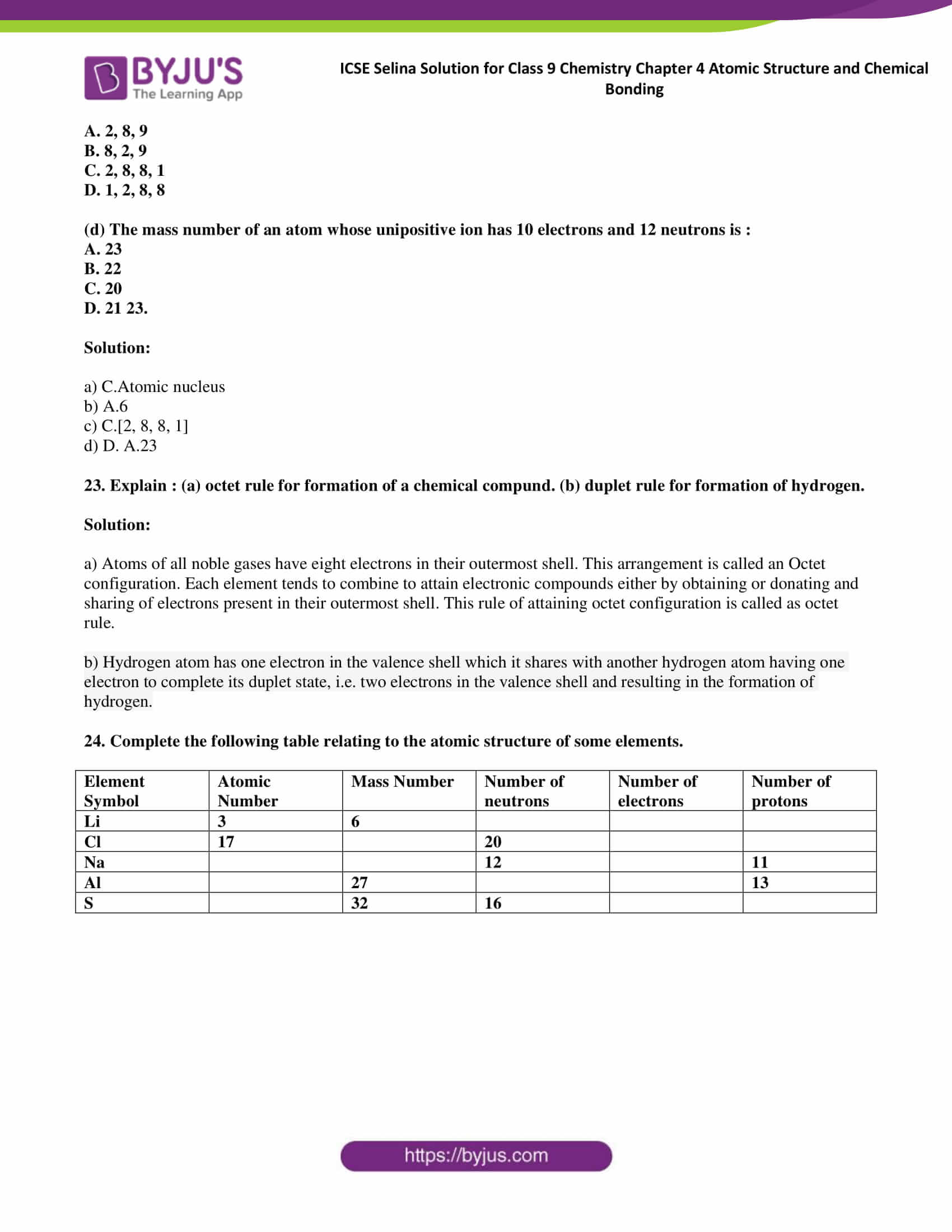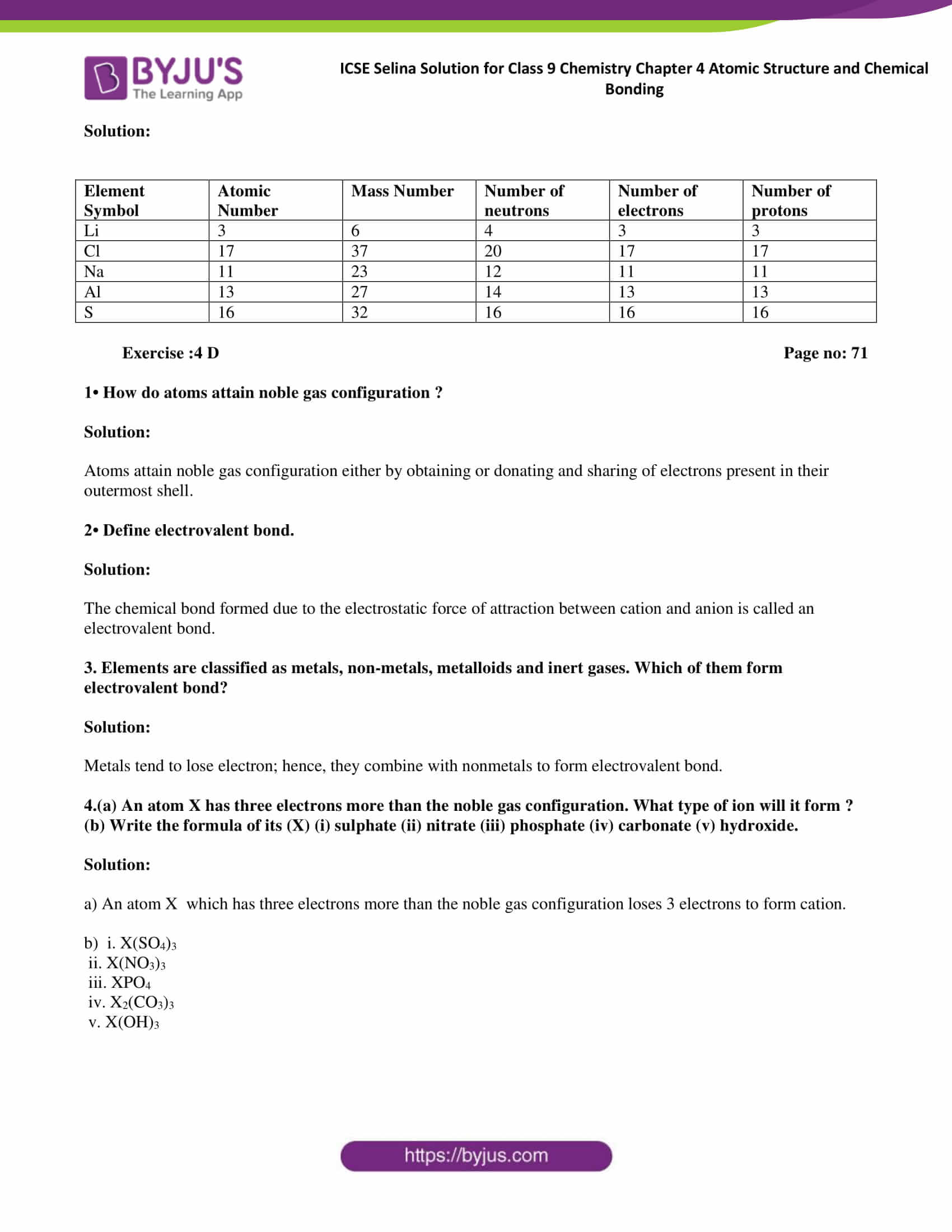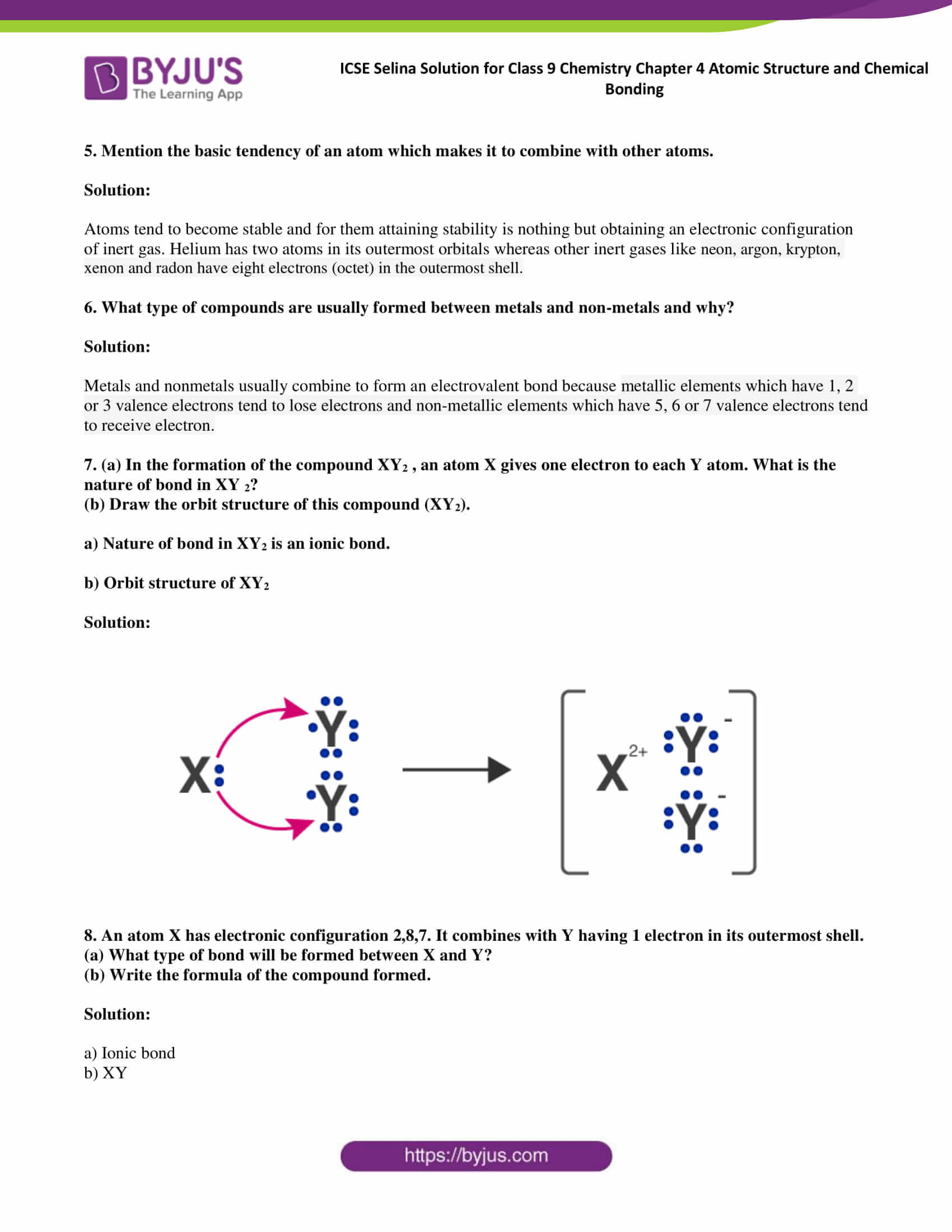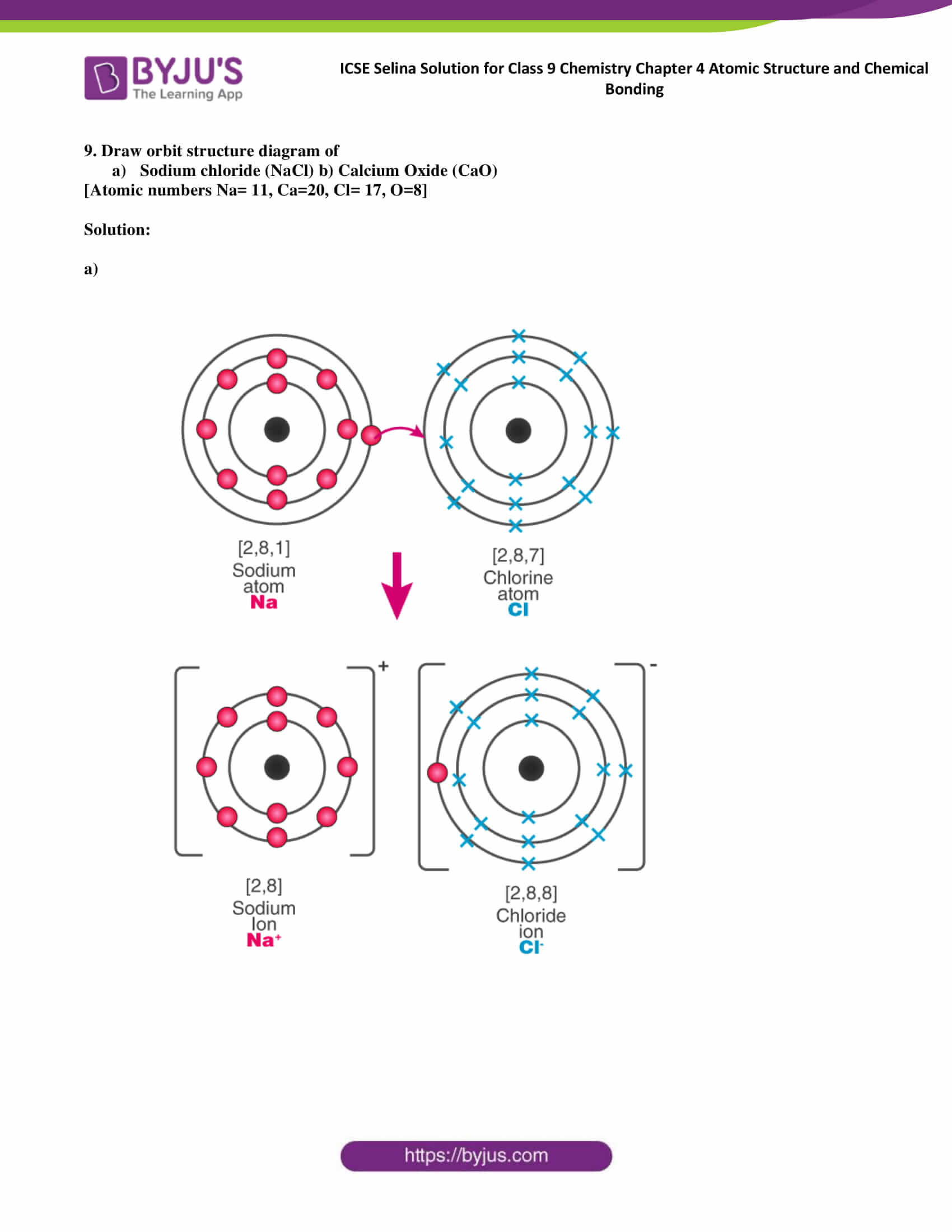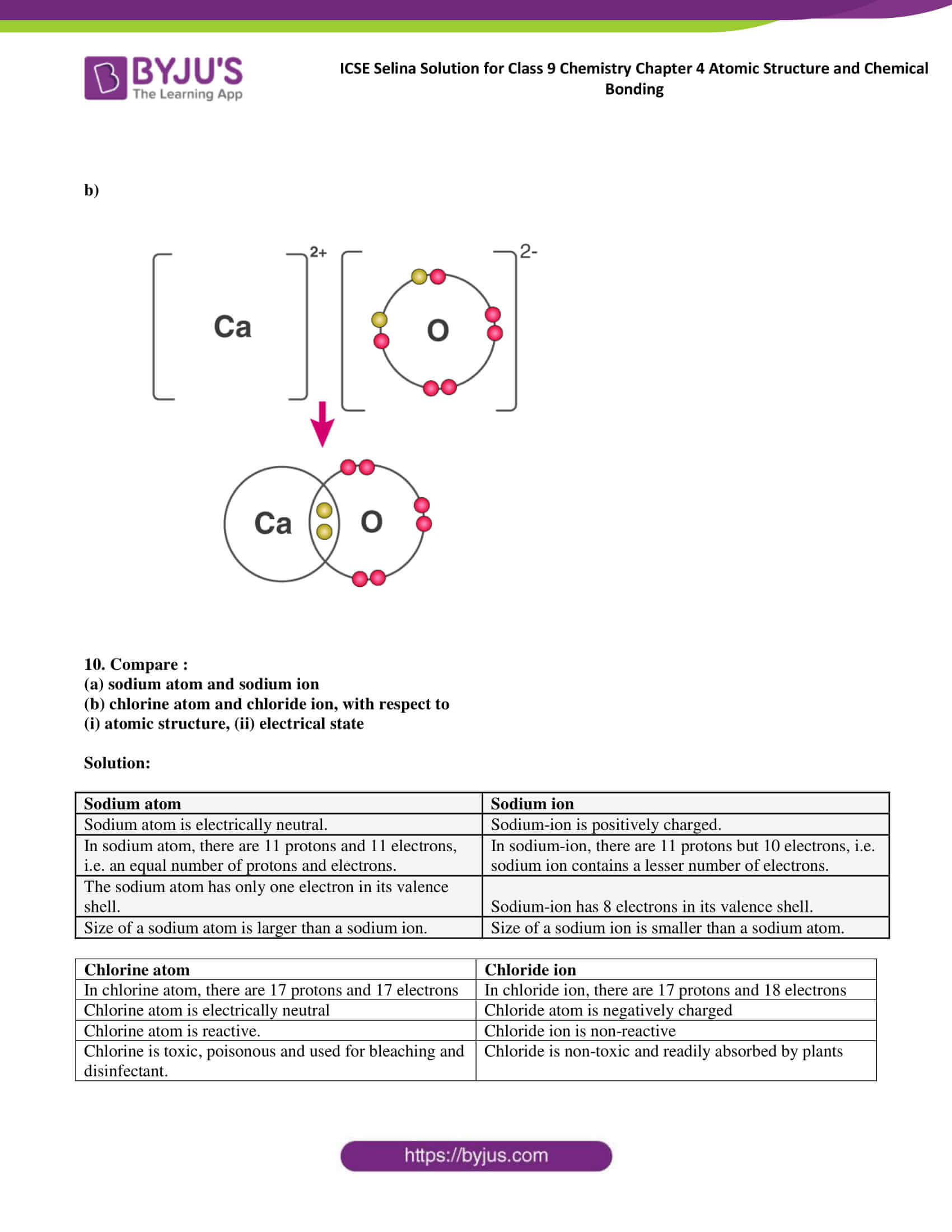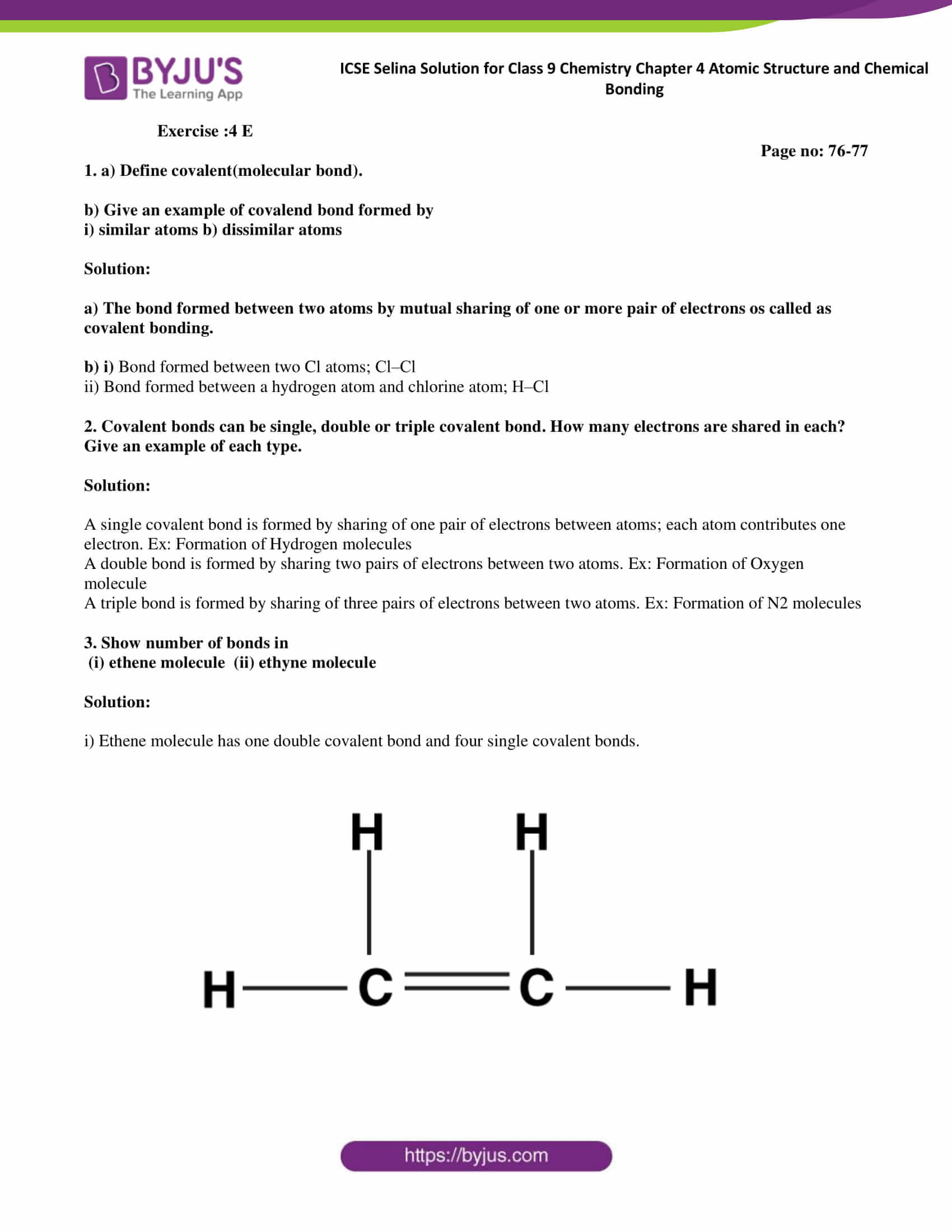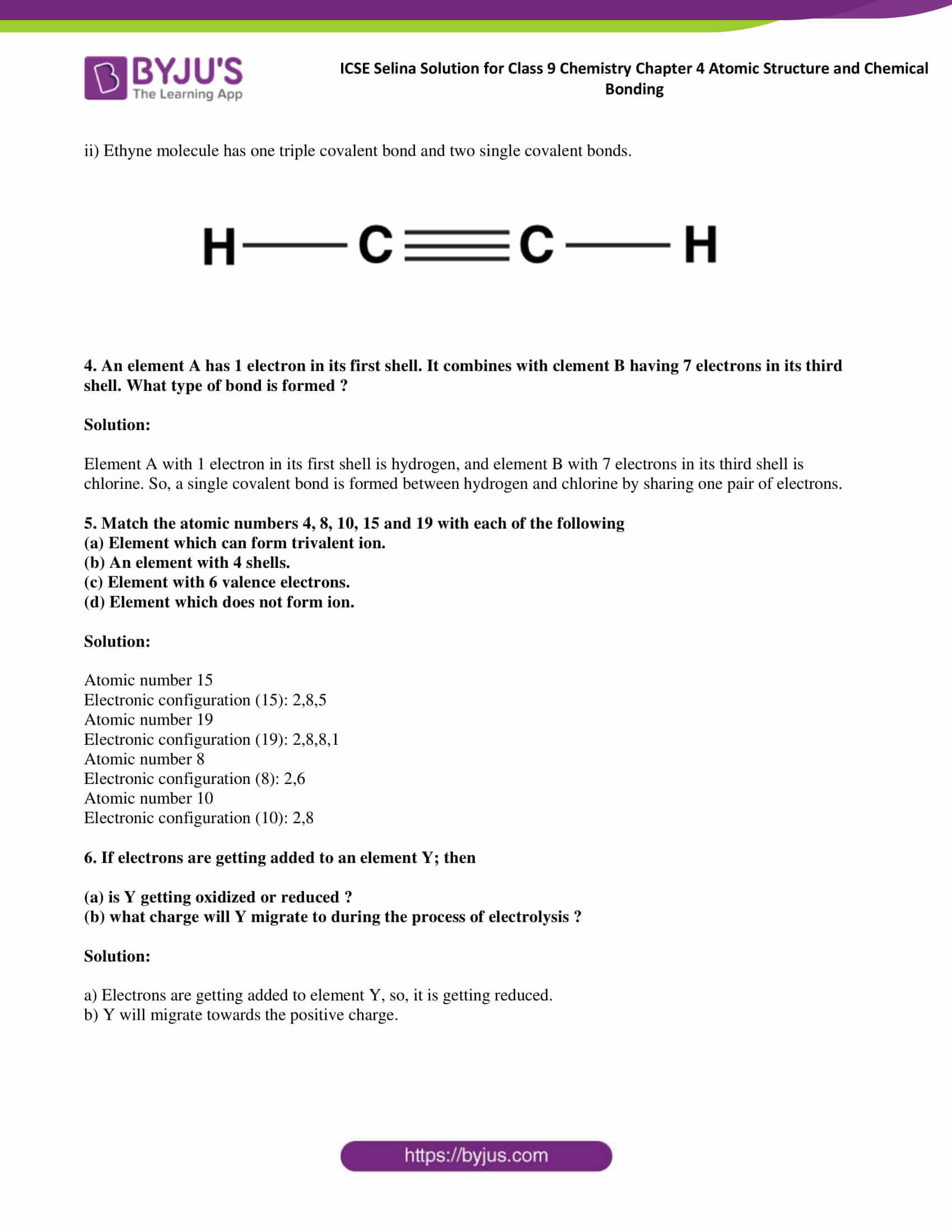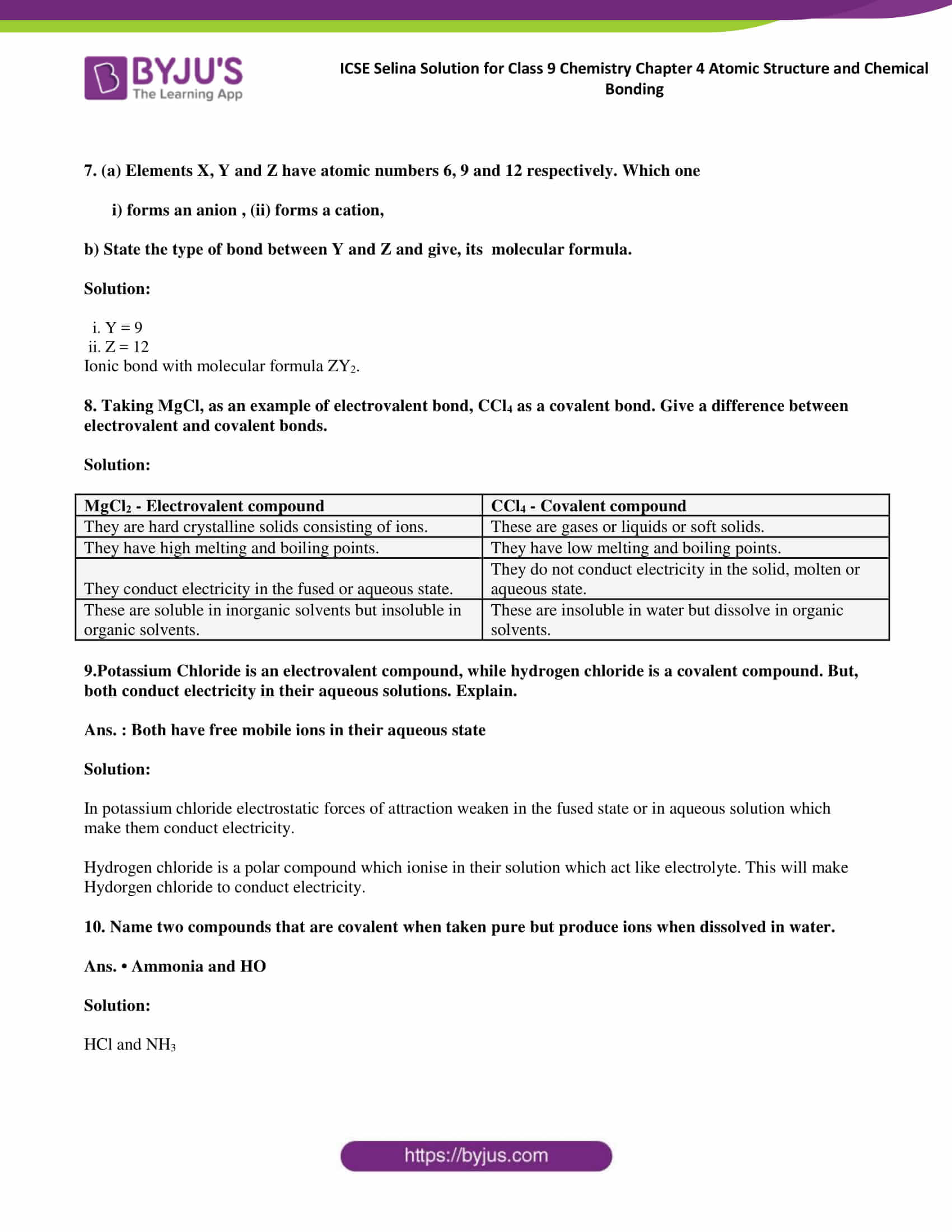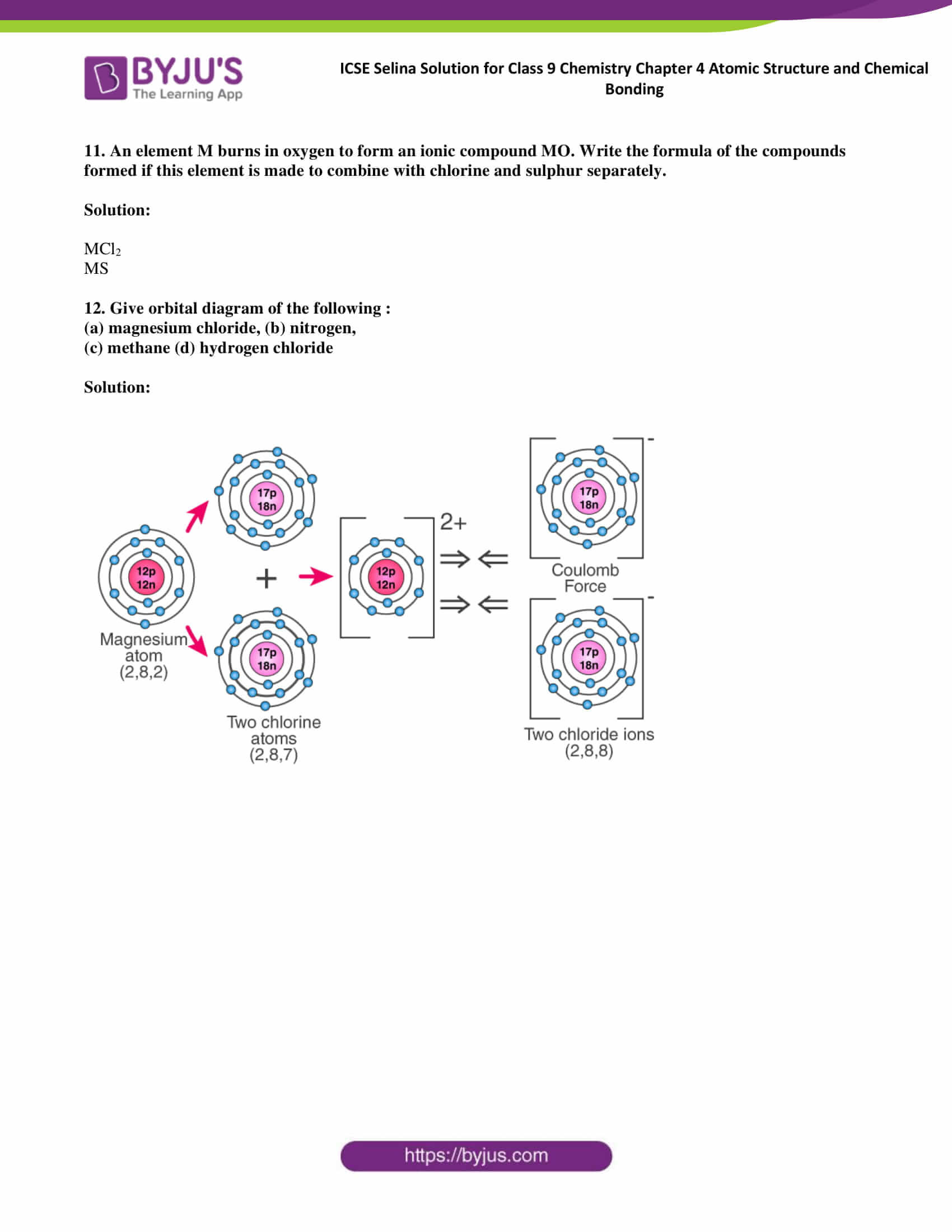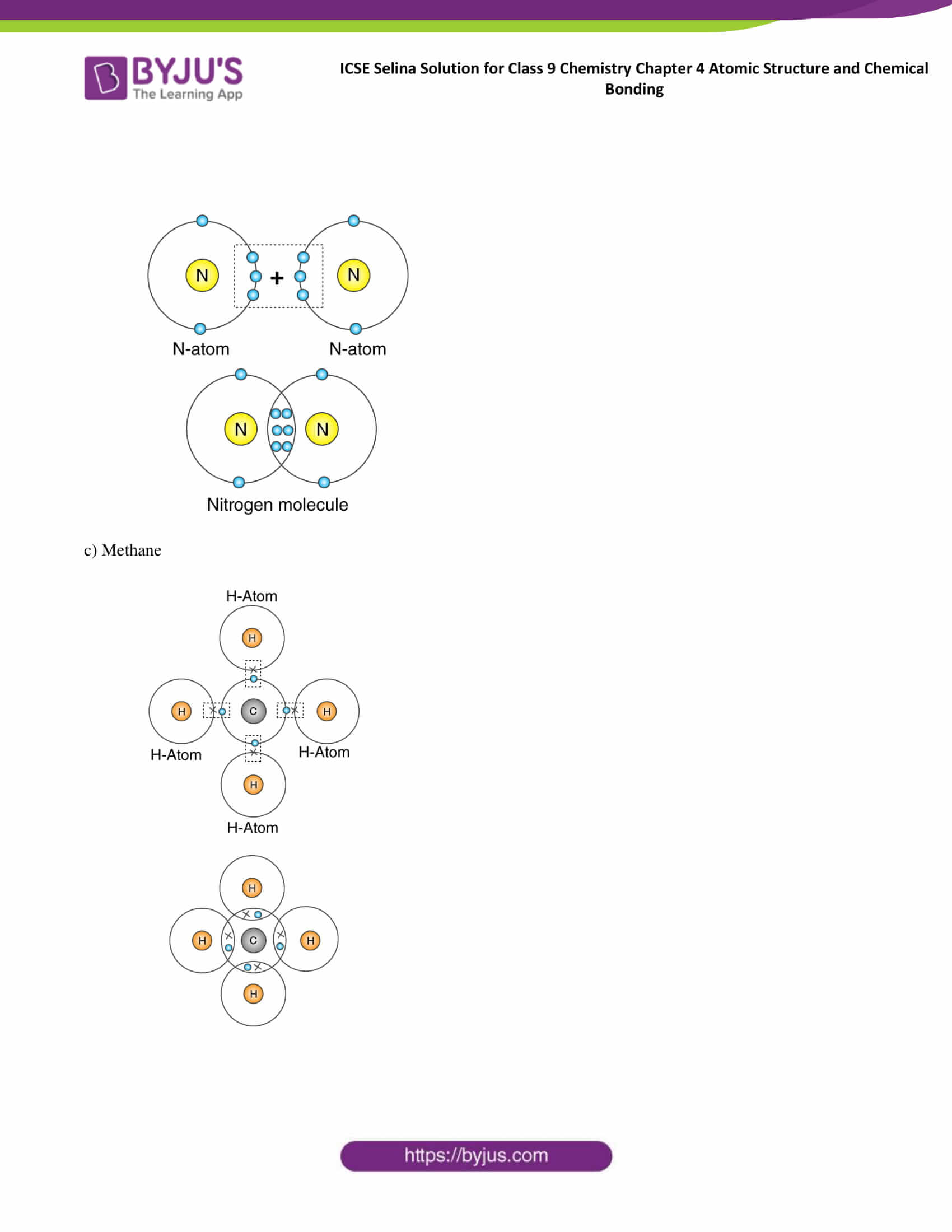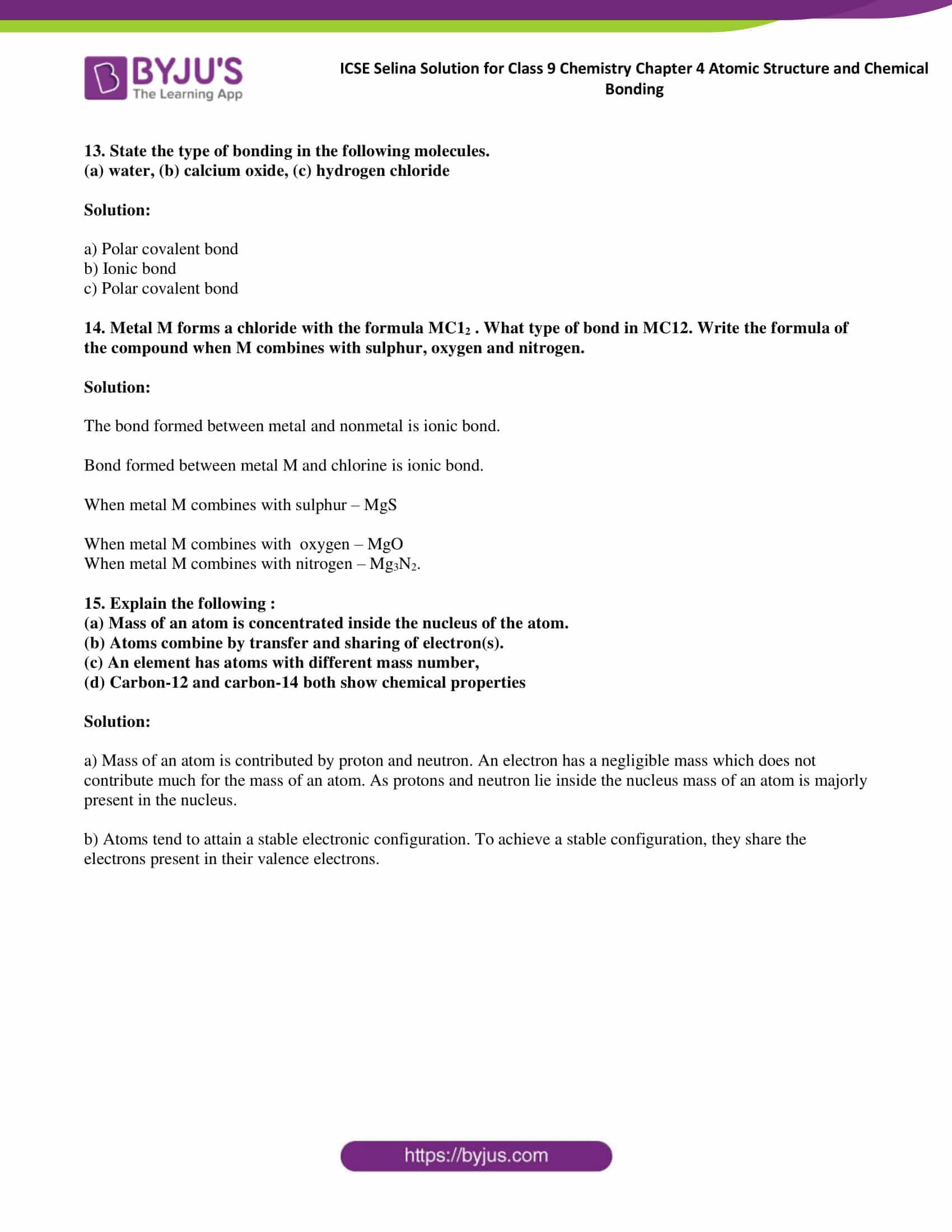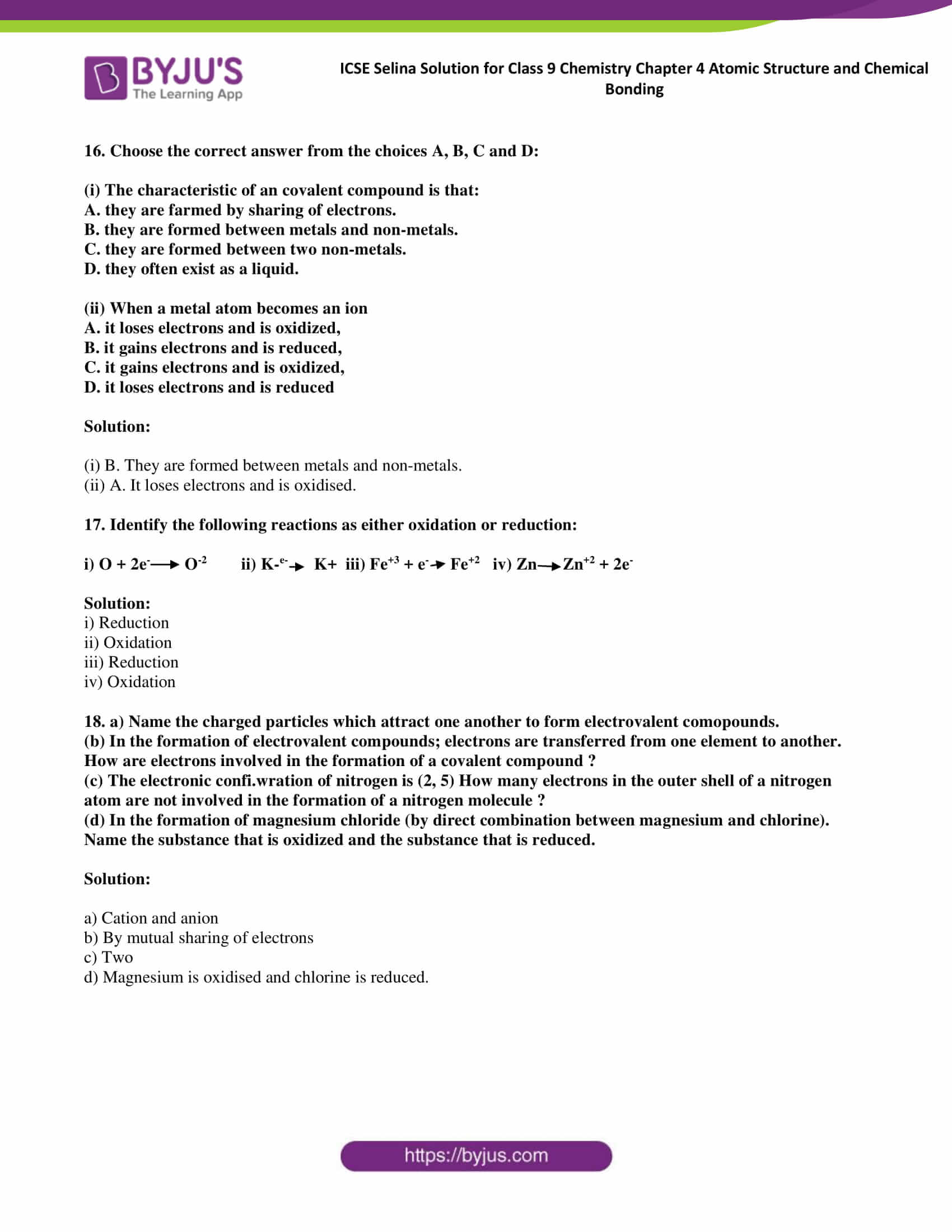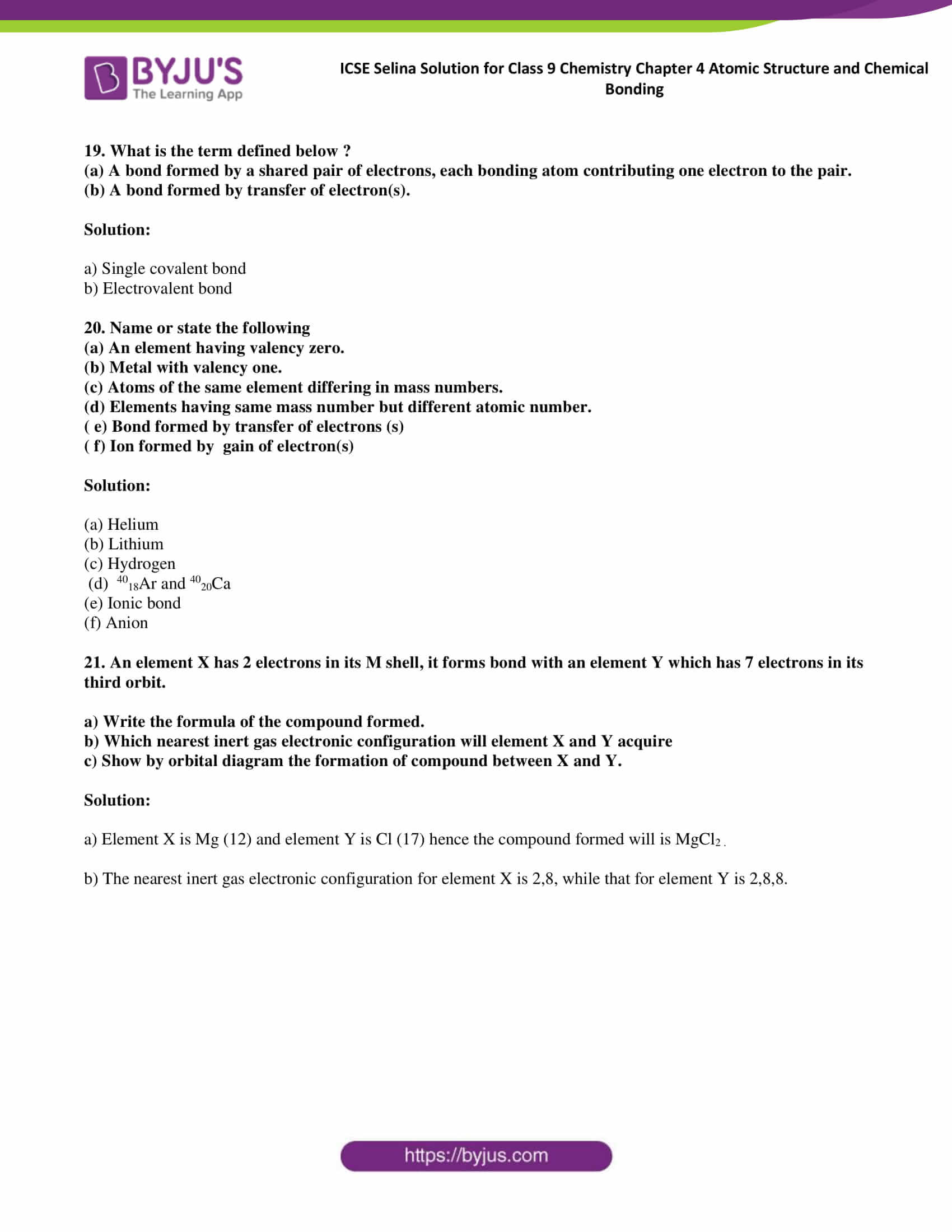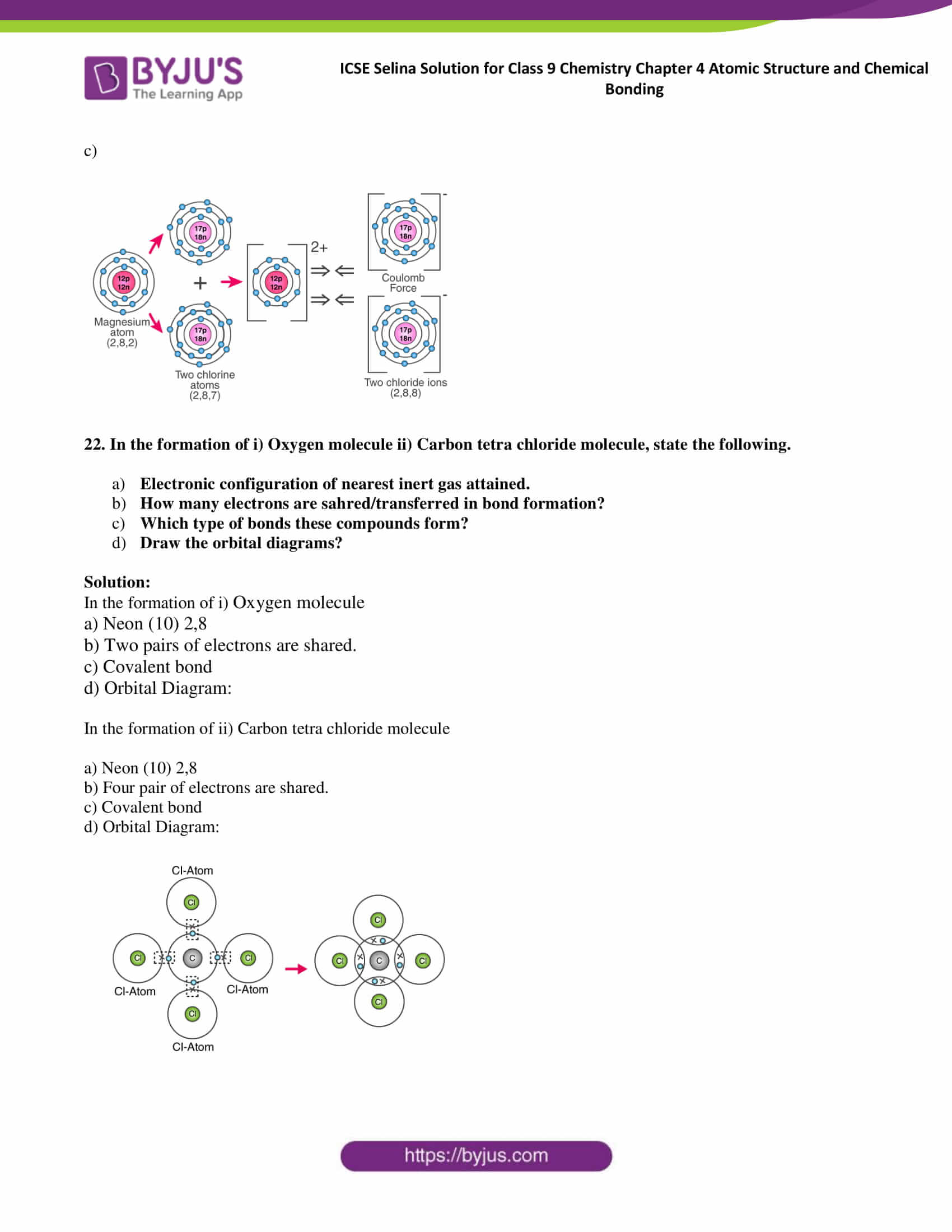### Access Answers to Selina publication ICSE Class 9 Chemistry Chapter 4 Atomic Structure and Chemical Bonding

Exercise:4 A

1. What is the contribution of the following in Atomic structure?

Solution:

a) Maharshi Kanada gave the idea of the smallest units of matter. According to him, matter consisted of indestructible minute particles called paramanus, which are now called atoms. He also gave the concept of the molecule.

b) Democritus called the paramanu as an atom, which is derived from the Greek word atomos, meaning indivisible.

2. State Dalton’s atomic theory.

Solution:

Dalton atomic theory states that atoms are indivisible particles which are the fundamental building blocks of matter. He states that the existence of different types of matter is due to different types of atoms.

Main postulates of Dalton’s atomic theory:

• Matter consists of very small and indivisible particles called atoms.
• Atoms can neither be created nor be destroyed.
• The atoms of an element are alike in all respects, but they differ from the atoms of other elements.
• Atoms of an element combine in small numbers to form molecules.
• Atoms of one element combine with atoms of another element in a simple ratio to form molecules of compounds.
• Atoms are the smallest units of matter which can take part in a chemical reaction.

3. What is an α (alpha) particle?

Solution:

A doubly charged helium ion (He2+) containing two protons and two neutrons is called an α-particle. α (alpha) particle is formed by removing two electrons from the helium atom.

4. What are the cathode rays? How are these rays formed?

Solution:

Rays that originate from the negative plate (Cathode) and travel towards anode are called cathode rays.

Formation of cathode rays

When a high voltage charge from an induction coil is applied to tubes filled with gases at very low pressure (0.01 mm of mercury), the gases become good conductors of electricity and begin to flow in the form of rays, which are cathode rays.

5. What is the nature of a charge on (i) cathode rays (ii) anode rays?

Solution:

Cathode rays are negatively charged as they are made of negatively charged particles.

Anode rays are positively charged as they are made of positively charged particles.

6. How are X-rays produced?

Solution:

X- rays are produced when a beam of cathode rays is made to fall upon hard metallic targets like tungsten.

7. Why were anode rays also called as ‘canal rays’?

Solution:

In discharge tube experiment set of rays travelling in a direction opposite to that of the cathode rays were found. These were called canal rays because they passed through the holes or canals in the cathode.

8. How do cathode rays differ from anode rays?

Solution:

 Cathode rays Anode rays They travel from the cathode to the anode. They travel from the anode to the cathode. They are made of negatively charged particles. They are made of positively charged particles. They produce a greenish-yellow fluorescence on a soda-glass screen. They produce fluorescence on a zinc sulphide screen. They are inflected towards a positive field and deflected towards a negative field. They are deflected by electric and magnetic fields towards positive fields.

9. State one observation which shows that atom is not indivisible.

Solution:

Chadwick discovered neutral particles present in an atom by bombarding light nuclei like beryllium with alpha particles. This observation shows that the atom is divisible.

10. a) Name an element which does not contain neutron.

b) If an atom contains one electron and one proton, will it carry any charge or not?

Solution:

a) Hydrogen does not contain neutron.

b) If an atom contains one electron and one proton, it will be neutral in charge.

11. On the basis of Thomson’s model of an atom, explain how an atom as a whole is neutral.

Solution:

According to Thomson’s atomic model, an atom is made of positively charged substances in the form of a sphere. Electrons are embedded into this sphere, and the total positive charge of the sphere is equal to the total negative charge of electrons, and hence, the atom remained electrically neutral.

12. Which sub-atomic particle was discovered by

(a) Thomson (b) Goldstein (c) Chadwick.

Solution:

a. Thomson discovered electrons.

b. Goldstein discovered protons.

13, Name the sub-atomic particle whose charge is

a) +1 (b) —1 (c) 0. 14.

Solution:

a. Proton

b. Electron

c. Neutron

14. (a) Which metal did Rutherford select for his a particle scattering experiment and why ?

(b) What do you think would be the observation of a-particle scattering experiment is carried out on

(i) heavy nucleus like platinum (ii) light nuclei like lithium.

Solution:

a) Rutherford used gold for his scattering experiment because gold is the most malleable metal and he wanted the thinnest layer as possible.

b) i) If metal with a heavy nucleus is used for a-particle scattering experiment observation would be same as the original experiment.

ii) If light metal like lithium is used for a-particle scattering experiment, then massive alpha particles will push the nucleus and may not be deflected back.

15. On the basis of Rutherford’s model of an atom, which subatomic particle is present in the nucleus of an atom?

Solution:

Based on Rutherford’s model of an atom, which subatomic particle is present in the nucleus is the proton.

16. Which part of the atom was discovered by Rutherford?

Solution:

Rutherford discovered the nucleus.

17. How was it shown that the atom has empty space?

Solution:

Rutherford performed an experiment by allowing a stream of alpha particles to pass through a very thin gold foil. He observed that alpha particles pass through the metal foil without deviating from their path. This shows that an atom contains a large empty space called nuclear space.

18. State one major drawback of Rutherford’s model.

Solution:

The comparison of electrons with planets in the solar system is the main drawback of Rutherford’s model. Thus Rutherford’s model failed to explain the stability of an atom.

19. In the figure given alongside :

(a) Name the shells denoted by A, B and C. Which A shell has the least energy?

(b) Name X and state the charge on it.

(c) The above sketch is of the model of an atom.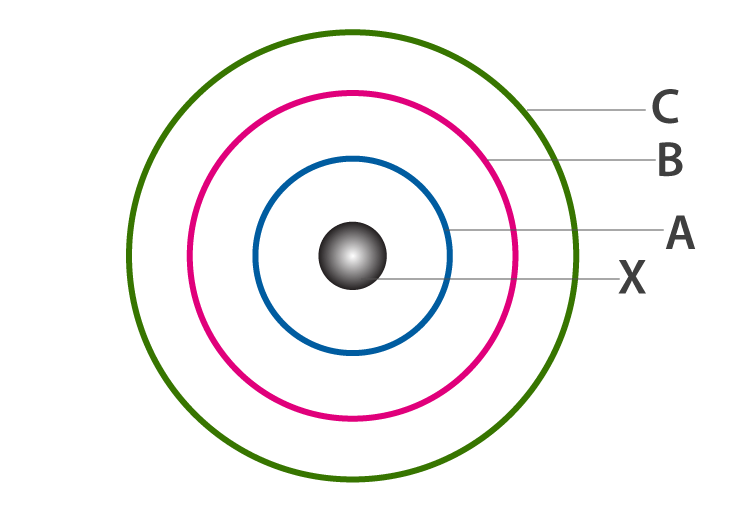Solution:

a)  A is for K shell or I shell.

B is for L shell or II shell.

C is for M shell or III shell.

Shell K has a minimum amount of energy.

b) X is a nucleus, it is positively charged.

c)  The above figure is of the Bohr model of an atom

20. Give the postulates of Bohr’s atomic model.

Solution:

Postulates of Bohr’s atomic model are as follows

i) The electrons revolving around the nucleus are confined to certain fixed orbits called shells or energy levels, each of which is associated with a fixed amount of energy.

ii) Electron neither loses nor gain electron while moving around the nucleus.

iii) An electron revolving in a particular orbit, on gaining a certain amount of energy, jumps to the next orbit and vice verse.

Exercise:4 B

1. (a) Name the three fundamental particles of an atom.

(b) Give the symbol and charge of each particle. 2, Complete the table given below by identifying P, Q, R and S.

Solution:

a) Electrons, protons and neutrons are the three fundamental particles of an atom.

b)

 Particle Symbol Charge Electron e -1 Proton P +1 Neutron n No charge

2. Complete the table given below by identifying P, Q and R

 Element Symbol No of Protons No of Neutrons No of Electrons Sodium $$\begin{array}{l}_{23}^{11}\textrm{Na}\end{array}$$ 11 P 11 Chlorine $$\begin{array}{l}_{35}^{17}\textrm{cl}\end{array}$$ Q 18 17 Uranium R 92 146 92 S $$\begin{array}{l}_{19}^{9}\textrm{Na}\end{array}$$ 9 10 9

Solution:

P- 12

Q-17

R-

$$\begin{array}{l}_{238}^{92}\textrm{U}\end{array}$$

3. The atom of an element is made up of 4 protons, 5 neutrons and 4 electrons. What are its atomic number and mass number ?

Solution:

Atomic number = Number of protons or number of electrons = 4

Mass number = Number of protons + Number of neutrons = 4 + 5 = 9

4. The atomic number and mass number of sodium are 11 and 23 respectively. What information is conveyed by this statement?

Solution:

Atomic number (11) of sodium conveys information that the number of protons and electrons is the same.

Mass number (23) of sodium indicates the sum of protons and neutrons.

5. Write down the names of the particles represented by the following symbols and explain the meaning of superscript and subscript numbers attached.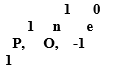Solution:

p = proton

n = neutron

e = electron

Top Superscript number: These superscript numbers show their mass number

Bottom Subscript number: These numbers show their atomic number

6. From the symbol

$$\begin{array}{l}_{24}^{12}\textrm{Mg}\end{array}$$
state the mass number, the atomic number and electronic configuration of magnesium.

Solution:

Mass number = 24

Atomic number = 12

No. of electrons = 24 – 12 = 12

Electronic configuration = 2, 8, 2

7. Sulphur has an atomic number 15 And mass number of 32.

State the number of protons and neutron in the nucleus of sulphur. Give a simple diagram to show the arrangement of electrons in an atom of sulphur.

Solution:

Atomic number = 16

Atomic mass = 32

Number of protons = 16

Number of electrons = 16

Number of neutrons = 32 – 16 = 16

Electronic configuration = 2, 8, 6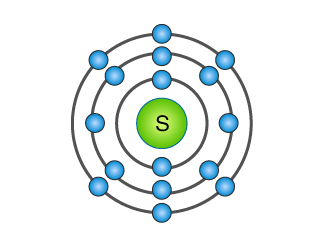8. Explain the rule according to which electrons are filled in various energy levels.

Solution:

i) The maximum capacity of a shell to accommodate electrons is given by the general formula 2n2, where n is the serial number of a shell.

ii) The maximum number of electrons possible in the outermost shell is 8, and that in the penultimate shell is 18.

iii) It is not necessary for an orbit to become completed before another is formed. In fact, a new orbit is formed when the outermost shell attains 8 electrons.

9. Draw the orbital diagram Ca+2 ion and state the number of three fundamental particles present in it

Solution: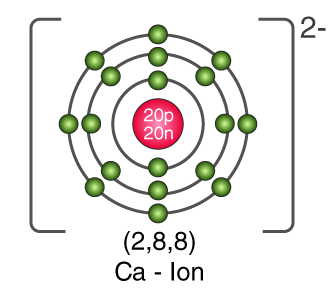Number of three fundamental particles of Ca2+:

Protons: 18

Electrons: 18

Neutrons: 40 – 18 = 22

10. Write down the electronic configuration of the following:

a)

$$\begin{array}{l}_{27}^{13}\textrm{X}\end{array}$$

b)

$$\begin{array}{l}_{35}^{17}\textrm{Y}\end{array}$$

Write down the number of electrons in X and neutrons in Y.

Solution:

1. Electronic configuration: 2, 8, 3
<Number of electrons = 13Number of neutrons= 27-13= 14
2. Electronic configuration: 2, 8, 7Number of electrons = 17Number of neutrons= 35-17= 18

Exercise:4 C

1. How does the Modern atomic theory contradict and correlate with Dalton’ s atomic theory?

Solution:

The latest research on the atom has proved that most of the postulates of Dalton’s atomic theory contradict. However, Dalton was right that atoms take part in chemical reactions.

Comparisons of Dalton’s atomic theory with the modern atomic theory.

Dalton’s atomic theory:

• Atoms are indivisible.
• Atoms of the same element are similar in every respect.
• Atoms combine in a simple whole-number ratio to form molecules.
• Atoms of different elements are different.
• Atoms can neither be created nor be destroyed.

Modern atomic theory:

• Atoms are divisible and consist of electrons, protons, neutrons and even more sub-particles.
• Atoms of the same element may differ from one another.
• Atoms of different elements may be similar called isobars.
• Atoms combine in a ratio which is not a simple whole-number ratio; e.g. in sugar, the C12H22O11 ratio is not a whole number ratio.

2. a) What are inert elements?

b) Why do they exist as monoatoms in molecules?

c) What is the valence electron?

Solution:

a) Elements whose outermost orbital is completely filled are known as inert elements.

b) Inert elements exist as monoatoms because molecules of these elements contain only one atom.

c) Electron present in the outermost orbital os known as valence electrons.

3. In what respect do the three isotopes of hydrogen differ ? Give their structures.

Solution:

The three isotopes of hydrogen differ in mass numbers which are 1,2 and 3 respectively known as protium, deuterium and tritium.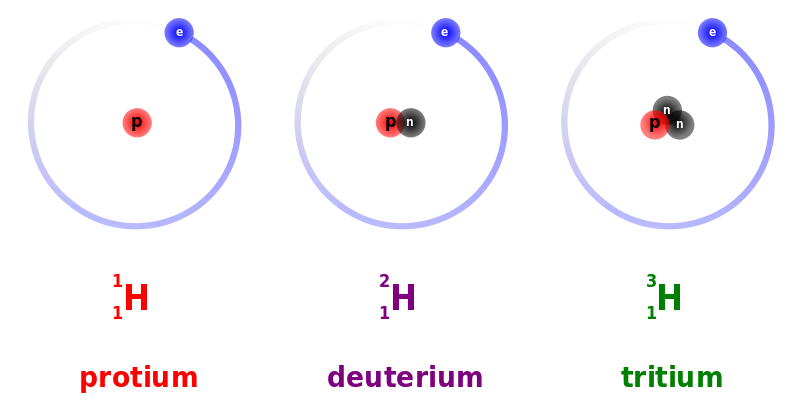4. Match the atomic numbers 4. 14. 8. 15. and 19 with each of the following :

(a) A solid non-metal of valency 3.

(b) A gas of valency 2.

(c) A metal of valency 1.

(d) A non-metal of valency 4.

Solution:

 Name with valency Atomic Number (a) A solid non-metal of valency 3. 15 (b) A gas of valency 2. 8 (c) A metal of valency 1. 19 (d) A non-metal of valency 4. 14

5. Draw diagrams representing the atomic structures of the following

(a) Sodium atom (b) Chloride ion

(c) Carbon atom (d) Oxide ion.

Solution: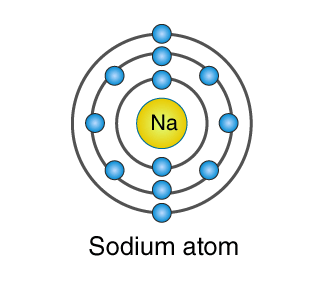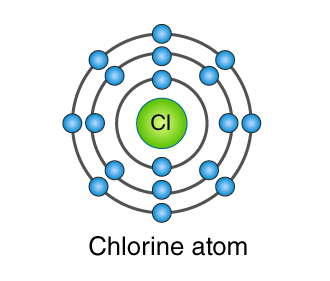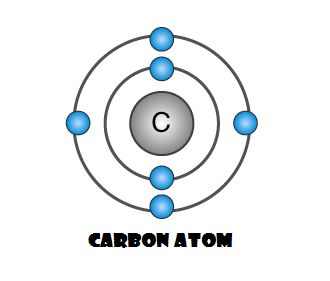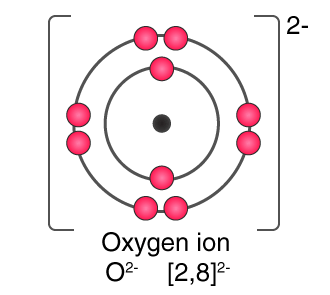6. What is the significance of the number of protons found in the atoms of different elements?

Solution:

The number of protons in the nucleus of an atom determines the element type of the atom.

7. Elements X, Y and Z have atomic numbers 6, 9 and 12 respectively. Which one :

(a) forms an anion (b) forms a cation

(c) has four electrons in its valence shell?

Solution:

a) Y(2, 7) forms an anion.

b) Z(2, 8, 2) forms a cation.

c) X(2, 4) has four electrons in the valence shell.

8. Element X has electronic configuration 2, 8, 18, 8, 1. Without identifying X,

1. Predict the sign and charge on a simple ion of X.
2. Write if X will be an oxidising agent or reducing agent. Why?

Solution:

a) X1+

b) The oxidising agent, because it can donate electrons.

9. Define the terms :

a) mass number (b) ion c) cation

d) anion (e) element f) orbit.

Solution:

a) The mass number is the sum of protons and neutrons present in the nucleus of an atom.

b) An ion is an atom carrying charge due to gaining or losing electrons

c) Positively charged ion which is formed after losing an electron is called a cation.

d) Negatively charged ion formed after gaining an electron is called anion

e) An element is a substance which cannot be split up into two or more simple substances by usual chemical methods of applying heat, light or electric energy

f) The circular path around the nucleus in which electrons of the atom revolve.

10. From the symbol

$$\begin{array}{l}_{4}^{2}\textrm{He}\end{array}$$
for the element helium, write down the mass number and the atomic number of the element

Solution:

Atomic number = 2

Mass number = 4

11. Five atoms are labelled A to E.

 Atoms Mass no Atomic no A 40 20 B 19 9 C 7 3 D 16 8 E 14 7

(a) Which one of these atoms:

(i) contains 7 protons

(ii) has electronic configuration 2 7.

(b) Write down the formula of the compound forme: between C and D.

(c) Predict : (i) metals (ii) non-metals.

Solution:

a.

Atom E contains 7 protons.

Atom B has an electronic configuration 2, 7.

b. Atom C stands for 7Li3, Atom D stands for 8O16.

Hence, the compound formula is Li2O.

c. Metals: A and C, Non-metals: B, D, E

12. An atom of an element has two electrons in the M she What is the (a) atomic number (b) number of protons in this element?

Solution:

Number of electrons in the M shell = 2

hence, the number of electrons in the K and L shells will be = 2, 8

Hence, atomic number = 2 + 8 + 2 = 12

Number of protons = 12

13.

$$\begin{array}{l}_{24}^{12}\textrm{Mg}\end{array}$$
and
$$\begin{array}{l}_{26}^{12}\textrm{Mg} \end{array}$$
are symbols of two isotopes of magnesium

(a) Compare the atoms of these isotopes with respect to :

(i) the composition of their nuclei.

(ii) their electronic configurations.

(b) Give reasons why the two isotopes of magnesium have different mass numbers.

Solution:

a)

i)

 $$\begin{array}{l}_{24}^{12}\textrm{Mg}\end{array}$$ $$\begin{array}{l}_{26}^{12}\textrm{Mg} \end{array}$$ No. of electrons 12 12 No. of protons 12 12 No. of neutrons 24 – 12 = 12 26-12 = 14

Hence, composition of nuclei

12Mg24 →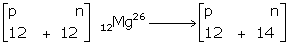ii). Electronic configuration = 2, 8, 2

b) Mass numbers of isotopes of Magnesium are different because of the different number of neutrons, i.e. 12 and 14, respectively.

14. What are nucleons? How many nucleons are present in phosphorus? Draw its structure.

Solution:

Constituents of the nucleus are called nucleons, i.e. protons and neutrons.

The atomic mass of phosphorus = 31

Atomic number = 15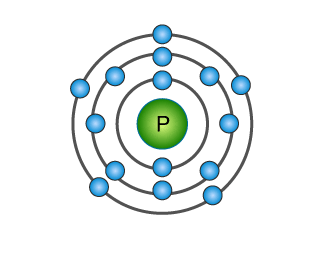15. What are isotopes? With reference to which fundamental particle do isotopes differ ? Give two uses of isotopes.

Solution:

Isotopes are the elements having the same atomic number but different mass numbers. Isotopes differ concerning neutrons.

Uses of isotopes

• Some isotopes are radioactive, and they are used to treat cancer Ex: cobalt isotopes
• An isotope of 235U is used as a fuel in a nuclear reactor.

16. Why do

$$\begin{array}{l}_{35}^{17}\textrm{Cl} \end{array}$$
and
$$\begin{array}{l}_{37}^{17}\textrm{Cl} \end{array}$$
have the same chemical properties? In what respect do these atoms differ?

Solution:

Electrons are the particles which take place in reaction. Chemical properties depend on the electronic configuration. In isotopes of

$$\begin{array}{l}_{35}^{17}\textrm{Cl} \end{array}$$
and
$$\begin{array}{l}_{37}^{17}\textrm{Cl} \end{array}$$
the atomic numbers are the same; hence, their electronic configuration remains the same, and so are their chemical properties.

These differ only in physical contents and weights because neutrons contribute to the mass of an atom which is 35 and 37 in this case.

17. Explain fractional atomic mass. What is the fractional mass of chlorine?

Solution:

Fractional atomic mass is the weighted average of all the naturally occurring isotopes of that element. Fractional mass of chlorine is 35.5.

18. a) What is meant by “ atomic number of an element”?

b) Complete the table given below:

 No of protons No of electrons No of neutrons Atomic number Mass number

Write down the electronic configuration of i) Chlorine atom ii) Chloride ion

Solution:

1. The number of protons in the nucleus of an atom represents atomic number of an element.

b)

 No of protons No of electrons No of neutrons Atomic number Mass number 17 17 18 17 35 17 17 20 17 37

c) i. Electronic configuration of chlorine = 2, 8, 7

ii. Electronic configuration of chlorine ion = 2, 8, 8

19. Name the following:

(a) The element which does not contain any neutron in its nucleus.

(b) An element having valency `zero’.

(c) Metal with valency

(d) Two atoms having the same number of protons and electrons but a different number of neutrons.

(e) The shell closest to the nucleus of an atom.

Solution:

a) Hydrogen

b) Helium

c) Magnesium

d) Hydrogen and carbon

e) K

20. Give reasons

(a) Physical properties of isotopes are different

(b) Argon does not react.

(c) Actual atomic mass is greater than mass number.

(d)

$$\begin{array}{l}_{35}^{17}\textrm{Cl} \end{array}$$
and
$$\begin{array}{l}_{37}^{17}\textrm{Cl} \end{array}$$
and do not differ in their chemical reactions.

Solution:

a) Physical properties are a dependent atomic mass of an element. Isotopes have different atomic mass hence they differ in their physical properties.

b) Arong outermost orbital is completly filled with 8 electrons. Hence, it does not react.

c) Actual atomic mass is greater than the mass number because the mass number is a whole number approximation of atomic mass unit.

d)

$$\begin{array}{l}_{35}^{17}\textrm{Cl} \end{array}$$
and
$$\begin{array}{l}_{37}^{17}\textrm{Cl} \end{array}$$
and do not differ in their chemical reactions their atomic mass determines the chemical reactivity. Here chlorine isotose have the same mass number.

21. An element A atomic number 7 mass number 14

B electronic configuration 2, 8, 8

C electrons 13, neutrons 14

D Protons 18 neutrons

E electronic configuration 2, 8, 8, 1

State (i) valency of each element (ii) which one is a metal (iii) which is a non-metal (iv) which is an inert gas.

Solution:

(i)

Element A

Atomic number = 7 = Number of electrons = 2, 5

Valency of A = 8 – 5 = 3

Element B

Electronic configuration 2, 8, 8

Valency of B = Zero

Element C has 13 electrons

Electronic configuration = 2, 8, 3

Valency of C = 3

Element D

Protons = 18 = Electrons = 2, 8, 8

Valency of D = Zero

Element E

Electronic configuration = 2, 8, 8, 1

Valency of E = 1

(ii) C and E are metals.

(iii) A is a non-metal.

(iv) A, C and E are not inert gases.

22. Choose the correct option

(a) Rutherford’s alpha-particle scattering experiment discovered

A. Electron

B. Proton

C. Atomic nucleus

D. Neutron

(b) Number of valence electrons in 0-2 is :

A. 6

B. 8

C. 10

D. 4

(c) Which of the following is the correct electronic configuration of potassium ?

A. 2, 8, 9

B. 8, 2, 9

C. 2, 8, 8, 1

D. 1, 2, 8, 8

(d) The mass number of an atom whose unipositive ion has 10 electrons and 12 neutrons is :

A. 23

B. 22

C. 20

D. 21 23.

Solution:

a) C.Atomic nucleus

b) A.6

c) C.[2, 8, 8, 1]

d) D. A.23

23. Explain : (a) octet rule for formation of a chemical compund. (b) duplet rule for formation of hydrogen.

Solution:

a) Atoms of all noble gases have eight electrons in their outermost shell. This arrangement is called an Octet configuration. Each element tends to combine to attain electronic compounds either by obtaining or donating and sharing of electrons present in their outermost shell. This rule of attaining octet configuration is called as octet rule.

b) Hydrogen atom has one electron in the valence shell which it shares with another hydrogen atom having one electron to complete its duplet state, i.e. two electrons in the valence shell and resulting in the formation of hydrogen.

24. Complete the following table relating to the atomic structure of some elements.

 Element Symbol Atomic Number Mass Number Number of neutrons Number of electrons Number of protons Li 3 6 Cl 17 20 Na 12 11 Al 27 13 S 32 16

Solution:

 Element Symbol Atomic Number Mass Number Number of neutrons Number of electrons Number of protons Li 3 6 4 3 3 Cl 17 37 20 17 17 Na 11 23 12 11 11 Al 13 27 14 13 13 S 16 32 16 16 16

Exercise :4 D

1• How do atoms attain noble gas configuration?

Solution:

Atoms attain noble gas configuration either by obtaining or donating and sharing of electrons present in their outermost shell.

2• Define electrovalent bond.

Solution:

The chemical bond formed due to the electrostatic force of attraction between cation and anion is called an electrovalent bond.

3. Elements are classified as metals, non-metals, metalloids and inert gases. Which of them form electrovalent bond?

Solution:

Metals tend to lose electron; hence, they combine with nonmetals to form electrovalent bond.

4.(a) An atom X has three electrons more than the noble gas configuration. What type of ion will it form?

(b) Write the formula of its (X) (i) sulphate (ii) nitrate (iii) phosphate (iv) carbonate (v) hydroxide.

Solution:

a) An atom X which has three electrons more than the noble gas configuration loses 3 electrons to form cation.

b)  i. X(SO4)3

ii. X(NO3)3

iii. XPO4

iv. X2(CO3)3

v. X(OH)3

5. Mention the basic tendency of an atom which makes it to combine with other atoms.

Solution:

Atoms tend to become stable and for them attaining stability is nothing but obtaining an electronic configuration of inert gas. Helium has two atoms in its outermost orbitals whereas other inert gases like neon, argon, krypton, xenon and radon have eight electrons (octet) in the outermost shell.

6. What type of compounds are usually formed between metals and non-metals and why?

Solution:

Metals and nonmetals usually combine to form an electrovalent bond because metallic elements which have 1, 2 or 3 valence electrons tend to lose electrons and non-metallic elements which have 5, 6 or 7 valence electrons tend to receive electron.

7. (a) In the formation of the compound XY2 , an atom X gives one electron to each Y atom. What is the nature of bond in XY 2?

(b) Draw the orbit structure of this compound (XY2).

a) Nature of bond in XY2 is an ionic bond.

b) Orbit structure of XY2

Solution: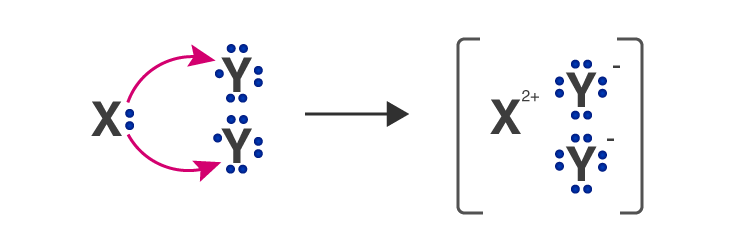8. An atom X has electronic configuration 2,8,7. It combines with Y having 1 electron in its outermost shell.

(a) What type of bond will be formed between X and Y?

(b) Write the formula of the compound formed.

Solution:

a) Ionic bond

b) XY

9. Draw orbit structure diagram of

1. Sodium chloride (NaCl) b) Calcium Oxide (CaO)

[Atomic numbers Na= 11, Ca=20, Cl= 17, O=8]

Solution:

a)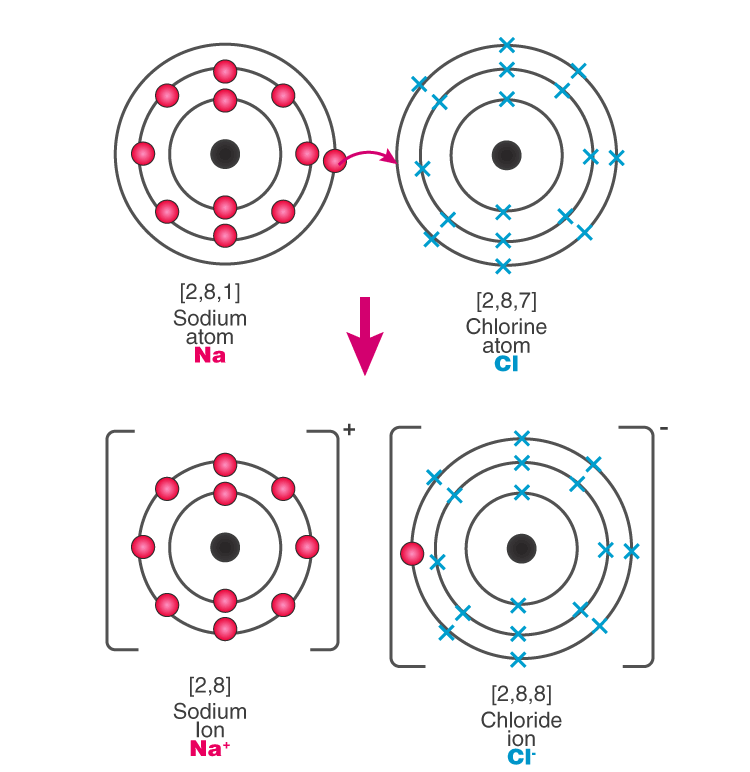b)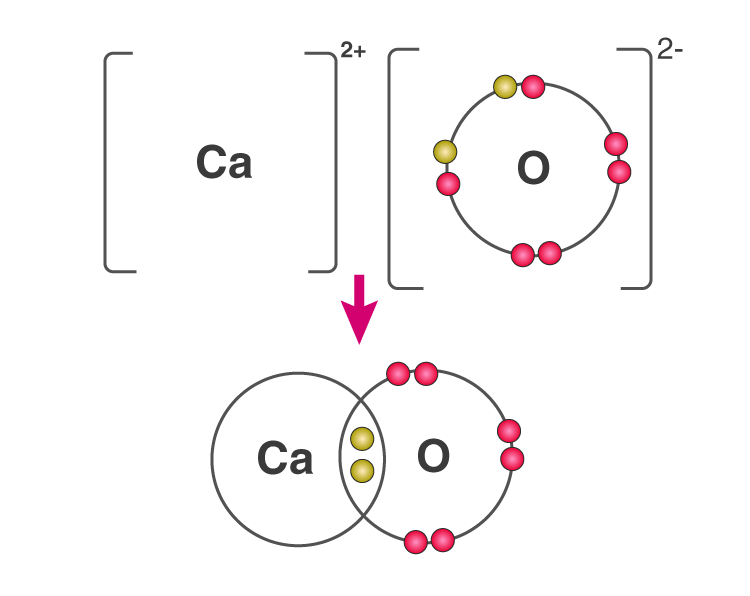10. Compare :

(a) sodium atom and sodium ion

(b) chlorine atom and chloride ion, with respect to

(i) atomic structure, (ii) electrical state

Solution:

 Sodium atom Sodium ion Sodium atom is electrically neutral. Sodium-ion is positively charged. In sodium atom, there are 11 protons and 11 electrons, i.e. an equal number of protons and electrons. In sodium-ion, there are 11 protons but 10 electrons, i.e. sodium ion contains a lesser number of electrons. The sodium atom has only one electron in its valence shell. Sodium-ion has 8 electrons in its valence shell. Size of a sodium atom is larger than a sodium ion. Size of a sodium ion is smaller than a sodium atom.
 Chlorine atom Chloride ion In chlorine atom, there are 17 protons and 17 electrons In chloride ion, there are 17 protons and 18 electrons Chlorine atom is electrically neutral Chloride atom is negatively charged Chlorine atom is reactive. Chloride ion is non-reactive Chlorine is toxic, poisonous and used for bleaching and disinfectant. Chloride is non-toxic and readily absorbed by plants

11. The electronic configuration of fluoride ion is the same as that of a neon atom. What is the difference between the two ?

Solution:

Fluoride ion is a negatively charged ion with 9 protons and 10 electrons, whereas Neon atom is electrically neutral with 10 protons and 10 electrons.

12. (a) What do you understand by redox reactions?

(b) Explain oxidation and reduction in terms of loss or gain of electrons.

Solution:

a) In redox reaction, there is the transfer of electrons, which results in the bond formation. The electropositive atom undergoes oxidation, while the electronegative atom undergoes reduction.

b) Oxidation is a process in which an atom or ion looses electrons. The reduction is a process in which an atom or ion gains electrons.

13. Potassium (at No. 19) and chlorine (at No. 17) form a compound. Explain the formation of the compound on the basis of (a) oxidation (b) reduction (c) oxidising agent (d) reducing agent.

Solution:

2K + Cl2 → 2KCl

a) Oxidation: In the electronic concept, oxidation is a process in which an atom or ion loses an electron(s).

K → K+ e

b) Reduction: In the electronic concept, reduction is a process in which an atom or ion accepts electron(s).

Cl2 + 2e→ 2Cl

c) Oxidising agent

An oxidising agent oxidises other substances either by accepting electrons or by providing oxygen or an electronegative ion, or by removing hydrogen or an electropositive ion.

Cl2 + 2e→ 2Cl

d) Reducing agent

A reducing agent reduces other substances either by providing electrons or by providing hydrogen or an electropositive ion, or by removing oxygen or an electronegative ion.

K → K+ e

Exercise :4 E Page no: 76-77

1. a) Define covalent(molecular bond).

b) Give an example of covalend bond formed by

i) similar atoms b) dissimilar atoms

Solution:

a) The bond formed between two atoms by mutual sharing of one or more pair of electrons os called as covalent bonding.

b) i) Bond formed between two Cl atoms; Cl‒Cl

ii) Bond formed between a hydrogen atom and chlorine atom; H‒Cl

2. Covalent bonds can be single, double or triple covalent bond. How many electrons are shared in each? Give an example of each type.

Solution:

A single covalent bond is formed by sharing of one pair of electrons between atoms; each atom contributes one electron. Ex: Formation of Hydrogen molecules

A double bond is formed by sharing two pairs of electrons between two atoms. Ex: Formation of Oxygen molecule

A triple bond is formed by sharing of three pairs of electrons between two atoms. Ex: Formation of N2 molecules

3. Show number of bonds in

(i) ethene molecule (ii) ethyne molecule

Solution:

i) Ethene molecule has one double covalent bond and four single covalent bonds.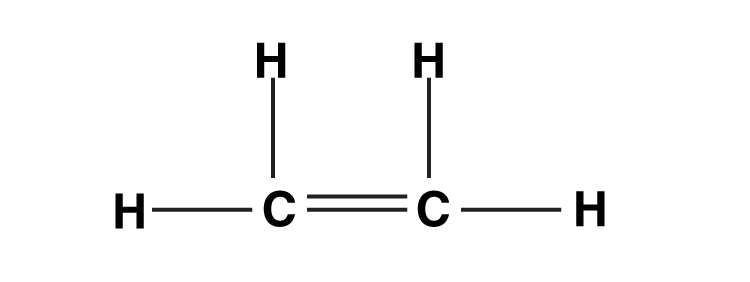ii) Ethyne molecule has one triple covalent bond and two single covalent bonds.4. An element A has 1 electron in its first shell. It combines with clement B having 7 electrons in its third shell. What type of bond is formed ?

Solution:

Element A with 1 electron in its first shell is hydrogen, and element B with 7 electrons in its third shell is chlorine. So, a single covalent bond is formed between hydrogen and chlorine by sharing one pair of electrons.

5. Match the atomic numbers 4, 8, 10, 15 and 19 with each of the following

(a) Element which can form trivalent ion.

(b) An element with 4 shells.

(c) Element with 6 valence electrons.

(d) Element which does not form ion.

Solution:

Atomic number 15

Electronic configuration (15): 2,8,5

Atomic number 19

Electronic configuration (19): 2,8,8,1

Atomic number 8

Electronic configuration (8): 2,6

Atomic number 10

Electronic configuration (10): 2,8

6. If electrons are getting added to an element Y; then

(a) is Y getting oxidized or reduced ?

(b) what charge will Y migrate to during the process of electrolysis ?

Solution:

a) Electrons are getting added to element Y, so, it is getting reduced.

b) Y will migrate towards the positive charge.

7. (a) Elements X, Y and Z have atomic numbers 6, 9 and 12 respectively. Which one

i) forms an anion , (ii) forms a cation,

b) State the type of bond between Y and Z and give, its molecular formula.

Solution:

i. Y = 9

ii. Z = 12

Ionic bond with molecular formula ZY2.

8. Taking MgCl, as an example of electrovalent bond, CCl4 as a covalent bond. Give a difference between electrovalent and covalent bonds.

Solution:

 MgCl2 – Electrovalent compound CCl4 – Covalent compound They are hard crystalline solids consisting of ions. These are gases or liquids or soft solids. They have high melting and boiling points. They have low melting and boiling points. They conduct electricity in the fused or aqueous state. They do not conduct electricity in the solid, molten or aqueous state. These are soluble in inorganic solvents but insoluble in organic solvents. These are insoluble in water but dissolve in organic solvents.

9.Potassium Chloride is an electrovalent compound, while hydrogen chloride is a covalent compound. But, both conduct electricity in their aqueous solutions. Explain.

Ans. : Both have free mobile ions in their aqueous state

Solution:

In potassium chloride electrostatic forces of attraction weaken in the fused state or in aqueous solution which make them conduct electricity.

Hydrogen chloride is a polar compound which ionise in their solution which act like electrolyte. This will make Hydorgen chloride to conduct electricity.

10. Name two compounds that are covalent when taken pure but produce ions when dissolved in water.

Ans. • Ammonia and HO

Solution:

HCl and NH3

11. An element M burns in oxygen to form an ionic compound MO. Write the formula of the compounds formed if this element is made to combine with chlorine and sulphur separately.

Solution:

MCl2

MS

12. Give orbital diagram of the following :

(a) magnesium chloride, (b) nitrogen,

(c) methane (d) hydrogen chloride

Solution: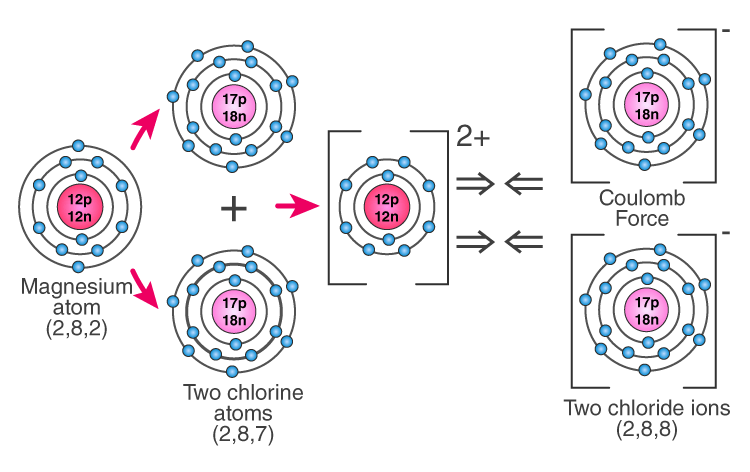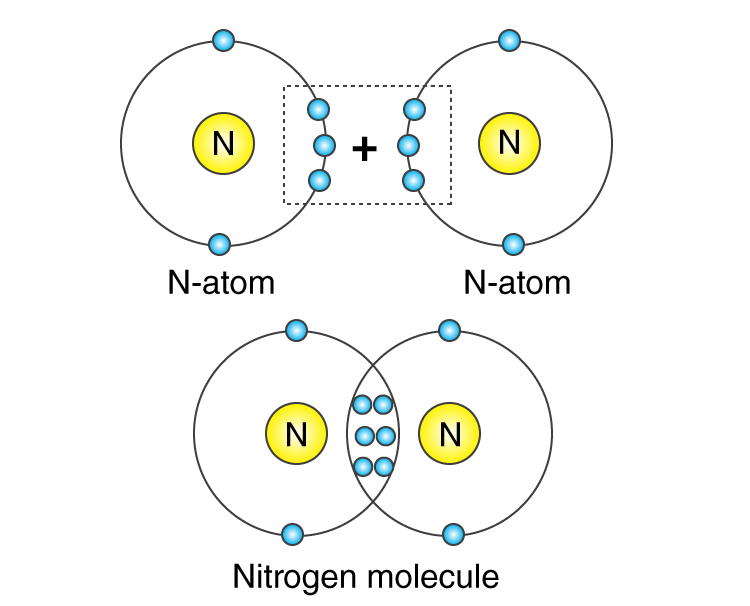c) Methane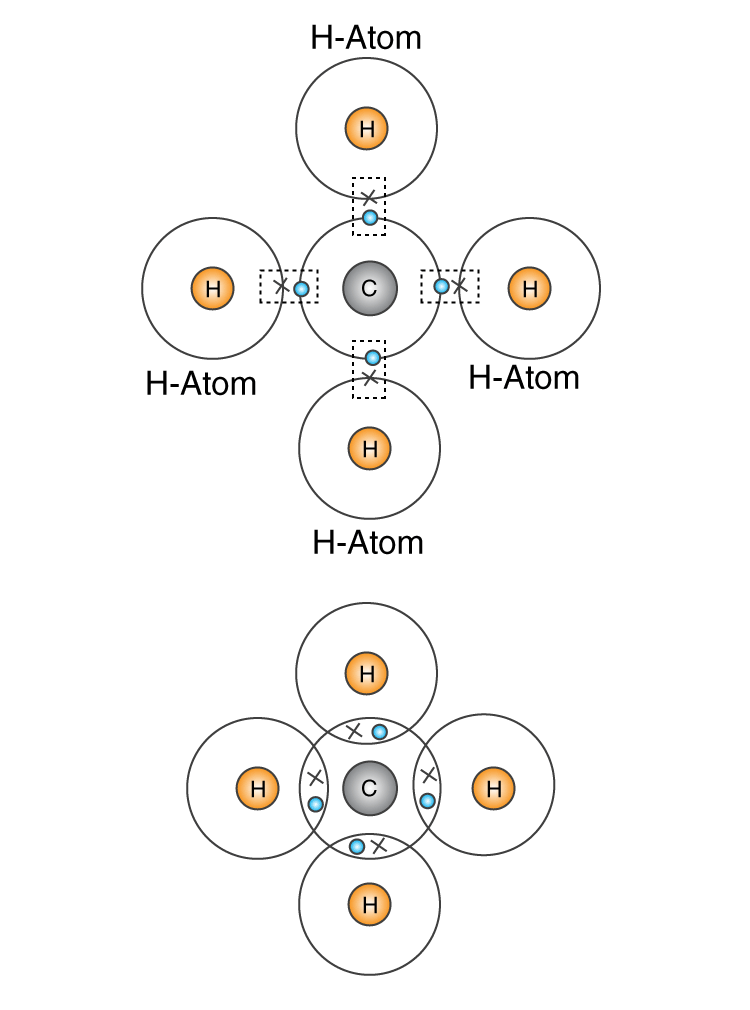13. State the type of bonding in the following molecules.

(a) water, (b) calcium oxide, (c) hydrogen chloride

Solution:

a) Polar covalent bond

b) Ionic bond

c) Polar covalent bond

14. Metal M forms a chloride with the formula MC12 . What type of bond in MC12? Write the formula of the compound when M combines with sulphur, oxygen and nitrogen.

Solution:

The bond formed between metal and nonmetal is ionic bond.

Bond formed between metal M and chlorine is ionic bond.

When metal M combines with sulphur – MgS

When metal M combines with  oxygen – MgO

When metal M combines with nitrogen – Mg3N2.

15. Explain the following :

(a) Mass of an atom is concentrated inside the nucleus of the atom.

(b) Atoms combine by transfer and sharing of electron(s).

(c) An element has atoms with different mass number,

(d) Carbon-12 and carbon-14 both show chemical properties

Solution:

a) Mass of an atom is contributed by proton and neutron. An electron has a negligible mass which does not contribute much for the mass of an atom. As protons and neutron lie inside the nucleus mass of an atom is majorly present in the nucleus.

b) Atoms tend to attain a stable electronic configuration. To achieve a stable configuration, they share the electrons present in their valence electrons.

16. Choose the correct answer from the choices A, B, C and D:

(i) The characteristic of an covalent compound is that:

A. they are farmed by sharing of electrons.

B. they are formed between metals and non-metals.

C. they are formed between two non-metals.

D. they often exist as a liquid.

(ii) When a metal atom becomes an ion

A. it loses electrons and is oxidized,

B. it gains electrons and is reduced,

C. it gains electrons and is oxidized,

D. it loses electrons and is reduced

Solution:

(i) B. They are formed between metals and non-metals.

(ii) A. It loses electrons and is oxidised.

17. Identify the following reactions as either oxidation or reduction:

i) O + 2e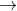O-2 ii) K-e-K+ iii) Fe+3+ e Fe+2 iv) ZnZn+2 + 2e

Solution:

i) Reduction

ii) Oxidation

iii) Reduction

iv) Oxidation

18. a) Name the charged particles which attract one another to form electrovalent comopounds.

(b) In the formation of electrovalent compounds; electrons are transferred from one element to another. How are electrons involved in the formation of a covalent compound ?

(c) The electronic confi.wration of nitrogen is (2, 5) How many electrons in the outer shell of a nitrogen atom are not involved in the formation of a nitrogen molecule ?

(d) In the formation of magnesium chloride (by direct combination between magnesium and chlorine). Name the substance that is oxidized and the substance that is reduced.

Solution:

a) Cation and anion

b) By mutual sharing of electrons

c) Two

d) Magnesium is oxidised and chlorine is reduced.

19. What is the term defined below?

(a) A bond formed by a shared pair of electrons, each bonding atom contributing one electron to the pair.

(b) A bond formed by transfer of electron(s).

Solution:

a) Single covalent bond

b) Electrovalent bond

20. Name or state the following.

(a) An element having valency zero.

(b) Metal with valency one.

(c) Atoms of the same element differing in mass numbers.

(d) Elements having same mass number but different atomic number.

( e) Bond formed by transfer of electrons (s)

( f) Ion formed by gain of electron(s)

Solution:

(a) Helium

(b) Lithium

(c) Hydrogen

(d)  4018Ar and 4020Ca

(e) Ionic bond

(f) Anion

21. An element X has 2 electrons in its M shell, it forms bond with an element Y which has 7 electrons in its third orbit.

a) Write the formula of the compound formed.

b) Which nearest inert gas electronic configuration will element X and Y acquire?

c) Show by orbital diagram the formation of compound between X and Y.

Solution:

a) Element X is Mg (12) and element Y is Cl (17) hence the compound formed will is MgCl2 .

b) The nearest inert gas electronic configuration for element X is 2,8, while that for element Y is 2,8,8.

c)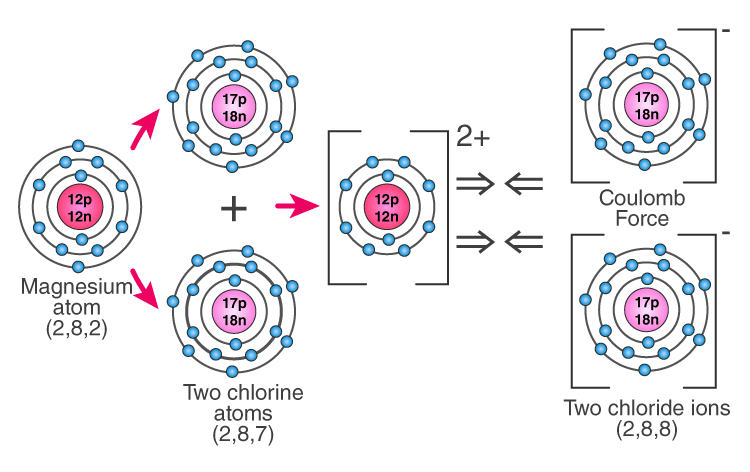22. In the formation of i) Oxygen molecule ii) Carbon tetra chloride molecule, state the following.

1. Electronic configuration of nearest inert gas attained.
2. How many electrons are sahred/transferred in bond formation?
3. Which type of bonds these compounds form?
4. Draw the orbital diagrams?

Solution:

In the formation of i) Oxygen molecule

a) Neon (10) 2,8

b) Two pairs of electrons are shared.

c) Covalent bond

d) Orbital Diagram:

In the formation of ii) Carbon tetra chloride molecule

a) Neon (10) 2,8

b) Four pair of electrons are shared.

c) Covalent bond

d) Orbital Diagram: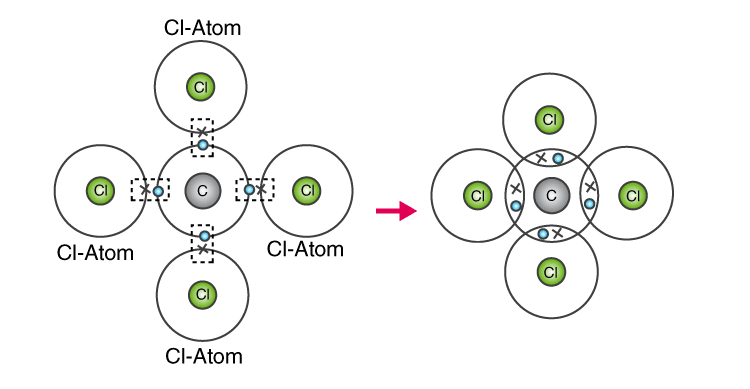For BYJU’S provides ICSE Books, ICSE Sample papers, Previous year question papers, Solved papers, and important question of ICSE Syllabus for all the subjects and classes Download BYJU’S Learning App.

## Frequently Asked Questions on Atomic Structure and Chemical Bonding

### State Dalton’s atomic theory?

Dalton atomic theory states that atoms are indivisible particles which are the fundamental building blocks of matter. He states that the existence of different types of matter are due to different types of atoms.

### What is an α (alpha) particle?

A doubly charged helium ion (He2+) containing two protons and two neutrons is called an α-particle. α (alpha) particle is formed by removing two electrons from the helium atom.

### What are cathode rays?

Rays that originate from the negative plate( Cathode) and travels towards anode are called cathode rays.

### How are X-rays produced?

X- rays are produced when a beam of cathode rays is made to fall upon hard metallic targets like tungsten.

### Why anode rays also called as ‘canal rays’?

In discharge tube experiment set of rays travelling in a direction opposite to that of the cathode rays were found. These were called canal rays because they passed through the holes or canals in the cathode.

### Which part of atom was discovered by Rutherford?

Rutherford discovered the nucleus.

### How was it shown that atom has empty space ?

Rutherford performed an experiment by allowing a stream of alpha particles to pass through a very thin gold foil. He observed that alpha particles pass through the metal foil without deviating from their path. This shows that an atom contains a large empty space called nuclear space.

### State one major drawback of Rutherford’s model?

The comparison of electrons with planets in the solar system is the main drawback of Rutherford’s model. Thus Rutherford’s model failed to explain the stability of an atom.